# AcuTrans

Translates the AcuSolve solution output to other formats.

## Syntax

acuTrans [options]

## Type

AcuSolve Post-Processing Program

## Description

The results of AcuSolve are stored using an internal format in a number of files in the directory specified by the working_directory option (ACUSIM.DIR by defaults) and in binary by default. AcuTrans is used to gather and translate these results into various formats more suited for post-processing or visualizing by third party products.

In the following, the full name of each option is followed by its abbreviated name and its type. For a general description of option specifications, see Command Line Options and Configuration Files. See below for more individual option details:

help or h (boolean)
If set, the program prints a usage message and exits. The usage message includes all available options, their current values and the place where each option is set.
problem or pb (string)
The name of the problem is specified via this option. This name is used to build internal file names and to generate output files. All generated output files start with the problem name.
working_directory or dir (string)
All internal files are stored in this directory. This directory does not need to be on the same file system as the input files of the problem.
run_id or run (integer)
Number of the run in which the translation is requested. If run_id is set to 0, the last run in the working directory is assumed.
translate_to or to (enumerated)
Translate the output to this format:
info
Prints information about the available time steps and variables.
table
Translate into a two dimensional array of data
stats
Print statistics on each output data
cgns
Translate to CGNS data base
ensight
Translate to EnSight 6.0 .case file
fieldview
Translate to FieldView unstructured binary file (2.4 or 2.7)
h3d
Translate to H3D format
ideas
Translate to I-deas Universal file
spectrum
Translate to Spectrum Visualizer compressed ASCII file
actran
Translate to ActranLA files
fieldview_options or fvopt (string)
Set FieldView translation options:
classical
Translate to combined mesh/solution unstructured binary file format 2.4
split
Translate to split file mesh/solution unstructured binary file format 2.7 to reduce disk file size
fieldview_region or fvr (enumerated)
Set FieldView region:
single
Create a single region
medium
Create one region per medium, that is, solid, fluid, shell
element_set
Create one region per element set
subdomain
Create one region per original subdomain
ensight_options or ensopt (string)
Set EnSight translation options:
6
Translate to EnSight 6
gold
Translate to EnSight Gold
h3d_options or h3dopt (string)
Set H3D translation options:
multi
Create separate files for the mesh and solutions at various time-steps
single
Create a single file
time_steps or ts (string)
Comma-separated list of time steps to be translated. The comma-separated fields have the general range format beg:end:inc, where :end:inc and :inc are optional. beg specifies the first time step in the range. It may be either a given time step, as specified by a number, the letter F (or f) requesting the first available time step, or the letter L (or l) requesting the last available time step. end is the last time step in the range. It may be either a time step number or L (or l) requesting the last available time step. If end is missing, the range is assumed to simply represent a single time step, that is, end=beg and inc=1. inc is the increment that ranges from beg to end. It may be either a number or the letter A (or a) requesting all available time steps in the range. If :inc is missing, it is assumed to be one. The range may also be specified by the single letter A (or a), requesting all available time steps. This is equivalent to F:L:A. time_steps is used only for nodal data and is ignored for time series data. Examples of time_steps option include:
acuTrans -ts 35            # step 35
acuTrans -ts 35,33,37       # steps 33, 35, and 37
acuTrans -ts 33:37:2        # steps 33, 35, and 37
acuTrans -ts 35,33:37:2,37  # steps 33, 35, and 37
acuTrans -ts 33:37          # all steps from 33 to 37
acuTrans -ts 33:37:A        # available steps from 33 to 37
acuTrans -ts F:L:A          # all available steps
acuTrans -ts A              # all available steps
optimization_case_time_steps or cts (string)
Comma-separated list of optimization case time steps to be translated. The comma-separated fields have the general range format beg:end:inc, where :end:inc and :inc are optional. beg specifies the first time step in the range. It may be either a given time step, as specified by a number, the letter F, or f, requesting the first available time step, or the letter L (or l) requesting the last available time step. end is the last time step in the range. It may be either a time step number or L (or l) requesting the last available time step. If end is missing, the range is assumed to simply represent a single time step, that is, end=beg and inc=1. inc is the increment that ranges from beg to end. It may be either a number or the letter A (or a) requesting all available time steps in the range. If :inc is missing, it is assumed to be one. The range may also be specified by the single letter A (or a), requesting all available time steps. This is equivalent to F:L:A.
optimization_case or case (string)
Comma-separated list of optimization cases to be translated.
Comma-separated list of runs to be translated. These are in addition to the main run given by run_id. Additional runs not compatible with the main are ignored. Combining four runs may be accomplished by:
acuTrans -run 1 -runs "2,3,4"
ignore_missing_steps or imts (boolean)
If set, missing requested time steps are ignored. Otherwise, if the requested time step does not exist, the command issues an error message and exits.
ignore_missing_cases or imcs (boolean)
If set, missing requested optimization cases are ignored. Otherwise, if the requested optimization case does not exist, the command issues an error message and exits.
ignore_missing_runs or imruns (boolean)
If set, missing runs are ignored. Otherwise, if the requested run does not exist, the command issues an error message and exits. This option is used with the -runs command line option.
ignore_missing_variables or imv (boolean)
If set, missing requested variables are ignored. Otherwise, if the requested variable does not exist, the command issues an error message and exits.
ignore_zeros or iz (boolean)
If set, ignore zeros when computing statistics, that is, when using -to stats. This option is usually used for variables that are defined only on surfaces. See extended_nodal_output.
mesh_output or mesh (boolean)
If set, the problem mesh is translated. This option is valid with translation formats ideas, spectrum, EnSight, H3D, FieldView and actran. Note that the CGNS formats always receive the mesh. When converting to FieldView and fvopt is set to single, the mesh is always translated.
remove_duplicate_surfaces or rmds (boolean)
If set, all surfaces are checked for uniqueness before being written into the CGNS, FieldView, EnSight, or H3D file. This prevents surfaces that are utilized multiple times in the input file for SIMPLE_BOUNDARY_CONDITION, SURFACE_OUTPUT, or ELEMENT_BOUNDARY_CONDITION commands from appearing multiple times in output files. This option reduces the size of output files and eliminates redundant boundary definitions. The option is on by default. You can recover pre-V12.0 behavior in AcuTrans by turning this option off.
mesh_movement or ale (boolean)
If set, output deformed coordinates for mesh_output. Otherwise, output the reference coordinates.
deformed_crd_type or defcrd (enumerated)
This option controls which displacement field is written to the mesh_displacement variable in all output types.
midstep
Write the mesh displacement field that corresponds to the displacement at the mid point of each time step. This option is useful when investigating the flow across non-conformal interfaces. The mid point of each time step is where the solution across the non-conformal interface is actually satisfied and should be visualized in order to see continuous contours across it. However, the boundary displacements for prescribed motions will also be written at the mid step and may not agree with the expectations based on input settings.
endstep
Write the mesh displacement field that corresponds to the displacement at the end point of each time step. This option produces a displacement field that aligns with the requested boundary displacements at each step, but does not correspond to the mesh displacement field that satisfies the governing equations. To visualize the mesh on which the governing equations are satisfied, use midstep. In practice, there is little use in this if there are no non-conformal interfaces present in the simulation and visualizing the solution on the mesh with the expected boundary displacements is preferred.
nodal_output or out (boolean)
If set, the nodal output, as specified by the NODAL_OUTPUT command in the input file, is translated. This option is valid with all translation formats.
nodal_output_vars or outv (string)
Comma-separated list of nodal_output variables to be translated. The list may include:
Table 1.
Variable (abbr) Fields Description
node_id (node) 1 User-given node number
coordinates (crd) 3 Nodal coordinates
density (dens) 1 Density, only for flow = compressible_navier_stokes.
velocity (vel) 3 Velocity vector
mach_number (mach) 1 Mach number, only for flow = compressible_navier_stokes.
pressure (pres) 1 Pressure
temperature (temp) 1 Temperature
relative_humidity 1 Relative humidity
dewpoint_temperature 1 Dewpoint temperature
humidity_film_thickness 1 Humidity film thickness
species (spec) nSpecs Species
field nFields Field values. For multi_field = levelset and multi_field = algebraic_eulerian, the field values correspond to volume fractions, and are named as volume_fraction-"fieldname". For multi_field = advective_diffusive, the field values correspond to mass fraction.
levelset (levelset) 1 Levelset
eddy_viscosity (eddy) 1 Turbulence eddy viscosity
kinetic_energy (tke) 1 Turbulence kinetic energy
eddy_frequency (tomega) 1 Turbulence eddy frequency
sqrt_eddy_period (tg) 1 Inverse of the square root of eddy frequency
dissipation_rate (teps) 1 Turbulence dissipation rate
intermittency (tintc) 1 Turbulence intermittency
transition_re_theta (treth) 1 Critical momentum thickness Reynolds Number
surface_y_plus (yp) 1 y+ on turbulence walls
surface_film_coefficient (film) 1 Convective heat transfer coefficient on turbulence walls
wall_shear_stress (wall_shear) 3 Wall shear stress on turbulence walls.
viscoelastic_stress (vest) 6 Viscoelastic stresses
mesh_displacement (mesh_disp) 3 Mesh displacement vector
mesh_velocity (mesh_vel) 3 Mesh velocity vector
where nSpecs is the number of species as given in the EQUATION command in the input file. Note that the problem must contain the requested variable in order for it to be translated. For example, the parameter turbulence in the EQUATION command must be set to a value other than none in order for eddy_viscosity to be available. The list of variables is sorted in the order given in the above table. If nodal_output_vars is set to _all, all available variables are translated. The surface_y_plus, surface_film_coefficient and wall_shear_stress are non-zero only on surface nodes given by TURBULENCE_WALL, or alternatively by SIMPLE_BOUNDARY_CONDITION of type wall. Note that the surface_film_coefficient is computed even if there is no temperature equation. However, all relevant fluid material models must include specific heat and conductivity models. It should also be noted that eddy_frequency and sqrt_eddy_period only appear when using the k-omega turbulence models. The dissipation_rate variable only appears when using the k-epsilon based turbulence models, and intermittency and transition_re_theta only appear when using the turbulence transition models.
extended_nodal_output or extout (boolean)
Extended nodal output flag. If set, adds to the nodal_output variable list available variables from running_average_output, time_average_output, derived_quantity_output, surface_output, radiation_surface, solar_radiation_surface, output_nodal_residual, output_error_estimator, and time_average_error_estimator. The nodal projections of miscellaneous element quantities and gradients of available field variables are also added to the list. Those variables defined only on a subset of the nodes, such as surfaces, are set to zero on the rest of the nodes. The list may include:
Table 2.
Variable (abbr) Fields Description
volume (vol) 1 Nodal volume
strain_rate_invariant_2 (strain_i2) 1 Second invariant of the strain rate tensor
velocity_magnitude (vmag) 1 Magnitude of the velocity vector
vorticity (vort) 3 Vorticity
cfl_number (cfl) 1 Element-integrated CFL number
density (dens) 1 Density
viscosity (visc) 1 Viscosity
material_viscosity (mat_visc) 1 Molecular viscosity
gravity (grav) 3 Gravity
specific_heat (cp) 1 Specific heat
conductivity (cond) 6 Conductivity
heat_source (heat_src) 1 Heat source
diffusivity (diff) nSpecs Species diffusivity
species_source (spec_src) nSpecs Species source
turbulence_y (turb_y) 1 Turbulence distance to wall
turbulence_y_plus (turb_yp) 1 Turbulence y+
des_length (deslen) 1 Length scale for DES turbulence model
field_diffusivity 1 Field diffusivity
field_source 1 Field source
material_conductivity 1 Material conductivity
total_pressure (tot_pres) 1 Total pressure
running_ave_velocity (ora_vel) 3 Running average velocity
running_ave_pressure (ora_pres) 1 Running average pressure
running_ave_temperature (ora_temp) 1 Running average temperature
running_ave_field (ora_field) nFields Running average field values. For multi_field = levelset and multi_field = algebraic_eulerian, the field values correspond to running average volume fractions, and are named as running_ave_volume_fraction-"fieldname".
running_ave_species (ora_spec) nSpecs Running average species
running_ave_eddy_viscosity (ora_eddy) 1 Running average eddy viscosity
running_ave_kinetic_energy (ora_tke) 1 Running average turbulence kinetic energy
running_ave_eddy_frequency (ora_tomega) 1 Running average turbulence eddy frequency
running_ave_sqrt_eddy_period (ora_tg) 1 Running average of inverse of the square root of eddy frequency
running_ave_dissipation_rate (ora_teps) 1 Running average turbulence dissipation rate
running_ave_intermittency (ora_tintc) 1 Running average of the turbulence intermittency
running_ave_transition_re_theta (ora_treth) 1 Running average of the critical momentum thickness Reynolds number
running_ave_mesh_displacement (ora_mesh_disp) 3 Running average mesh displacement
running_ave_viscoelastic_stress (ora_vest) 6 Running average viscoelastic stress
residual_velocity (onr_vel) 3 Residual of momentum equations
residual_pressure (onr_pres) 1 Residual of continuity equation
residual_temperature (onr_temp) 1 Residual of temperature equation
residual_species (onr_spec) nSpecs Residual of species equations
residual_eddy_viscosity (onr_eddy) 1 Residual of turbulence eddy viscosity equation
residual_kinetic_energy (onr_tke) 1 Residual of turbulence kinetic energy equation
residual_eddy_frequency (onr_tomega) 1 Residual of turbulence eddy frequency equation
residual_sqrt_eddy_period (onr_tg) 1 Residual of inverse of the square root of eddy frequency
residual_dissipation_rate (onr_teps) 1 Residual of turbulence dissipation rate equation
residual_intermittency (onr_tintc) 1 Residual of the turbulence intermittency
residual_transition_re_theta (onr_treth) 1 Residual of the critical momentum thickness Reynolds number
residual_mesh_displacement (onr_mesh_disp) 3 Residual of mesh displacement equations
residual_viscoelastic_stress (onr_vest) 6 Residual of viscoelastic stress
error_estimator_volume (oee_vol) 1 Volume
error_estimator_covariant_metric (oee_covar) 6 Covariant metric
error_estimator_velocity (oee_vel) 3 Error estimate of momentum equations
error_estimator_pressure (oee_pres) 1 Error estimate of continuity equation
error_estimator_temperature (oee_temp) 1 Error estimate of temperature equation
error_estimator_species (oee_spec) nSpecs Error estimate of species equations
error_estimator_eddy_viscosity (oee_eddy) 1 Error estimate of turbulence equation
error_estimator_viscoelastic_stress (oee_vest) 6 Error estimate of viscoelastic equations
error_estimator_tau_velocity (oee_tau_vel) 1 Error estimate of least-squares metric for continuity equation
error_estimator_tau_pressure (oee_tau_pres) 1 Error estimate of least-squares metric for momentum equations
error_estimator_tau_temperature (oee_tau_temp) 1 Error estimate of least-squares metric for heat equation
error_estimator_tau_species (oee_tau_spec) nSpecs Error estimate of least-squares metric for species equations
error_estimator_tau_eddy_viscosity (oee_tau_eddy) 1 Error estimate of least-squares metric for turbulence equations
error_estimator_tau_viscoelastic_stress (oee_tau_vest) 6 Error estimate of least-squares metric for viscoelastic equations
time_ave_error_volume (oae_vol) 1 Time-averaged volume
time_ave_error_covariant_metric (oae_covar) 6 Time-averaged covariant metric
time_ave_error_velocity (oae_vel) 3 Time-averaged error estimate of momentum equations
time_ave_error_pressure (oae_pres) 1 Time-averaged error estimate of continuity equation
time_ave_error_temperature (oae_temp) 1 Time-averaged error estimate of temperature equation
time_ave_error_species (oae_spec) nSpecs Time-averaged error estimate of species equations
time_ave_error_eddy_viscosity (oae_eddy) 1 Time-averaged error estimate of turbulence equation
time_ave_error_viscoelastic_stress (oae_vist) 6 Time-averaged error estimate of viscoelastic stress
time_ave_error_tau_velocity (oae_tau_vel) 1 Time-averaged error estimate of least-squares metric for continuity equation
time_ave_error_tau_pressure (oae_tau_pres) 1 Time-averaged error estimate of least-squares metric for momentum equations
time_ave_error_tau_temperature (oae_tau_temp) 1 Time-averaged error estimate of least-squares metric for heat equation
time_ave_error_tau_species (oae_tau_spec) nSpecs Time-averaged error estimate of least-squares metric for species equations
time_ave_error_tau_eddy_viscosity (oae_tau_eddy) 1 Time-averaged error estimate of least-squares metric for turbulence equation
time_ave_error_tau_viscoelastic_stress (oae_tau_vest) 6 Time-averaged error estimate of least-squares metric for viscoelastic equations
time_ave_velocity (ota_vel) 3 Time-averaged velocity
time_ave_velocity_square (ota_vel_sqr) 6 Time-averaged velocity square
time_ave_velocity_regular (ota_vel_reg) 3 Time-averaged non-conservative velocity
time_ave_pressure (ota_pres) 1 Time-averaged pressure
time_ave_pressure_square (ota_pres_sqr) 1 Time-averaged square of pressure
time_ave_stress (ota_stress) 6 Time-averaged Cauchy stress
surface_area (osf_area) 1 Surface area
surface_mass_flux (osf_mass) 1 Surface mass flux
surface_momentum_flux (osf_mom) 3 Surface momentum flux
surface_traction (osf_trac) 3 Surface traction
surface_moment (osf_moment) 3 Surface moment
surface_convective_temperature_flux (osf_conv_temp) 1 Surface convective temperature flux
surface_heat_flux (osf_heat) 1 Surface heat flux
surface_convective_species_flux (osf_conv_spec) nSpecs Surface convective species flux
surface_species_flux (osf_spec_flux) nSpecs Surface species flux
solar_area (oqf_area) 1 Solar area
solar_heat_flux (oqf_heat) 1 Solar heat flux
where nSpecs is the number of species as given in the EQUATION command in the input file. The output fields for time_ave_velocity_square and time_ave_stress are xx, yy, zz, xy, yz, and zx. The output fields for gradient variables are, for example, ux, uy, uz, vx,....
running_average_output or ora (boolean)
If set, the nodal running average field output, as specified by the RUNNING_AVERAGE_OUTPUT command in the input file, is translated. This option is valid with translation formats table and stats.
running_average_output_vars or orav (string)
Comma-separated list of running_average_output variables to be translated. The list may include:
Table 3.
Variable (abbr) Fields Description
node_id (node) 1 User-given node number
coordinates (crd) 3 Nodal coordinates
velocity (vel) 3 Velocity vector
pressure (pres) 1 Pressure
temperature (temp) 1 Temperature
relative_humidity 1 Relative humidity
dewpoint_temperature 1 Dewpoint temperature
humidity_film_thickness 1 Humidity film thickness
field nFields Fields. For multi_field = levelset and multi_field = algebraic_eulerian, the field values correspond to volume fractions, and are named as volume_fraction-"fieldname". For multi_field = advective_diffusive, the field values correspond to mass fraction.
species (spec) nSpecs Species
eddy_viscosity (eddy) 1 Turbulence eddy viscosity
kinetic_energy (tke) 1 Turbulence kinetic energy
eddy_frequency (tomega) 1 Turbulence eddy frequency
sqrt_eddy_frequency (sqrt_tg) 1 Inverse of the square root of eddy frequency
dissipation_rate (teps) 1 Turbulence dissipation rate
intermittency (tintc) 1 Turbulence intermittency
transition_re_theta (treth) 1 Critical momentum thickness Reynolds number
mesh_displacement (mesh_disp) 3 Mesh displacement vector
viscoelastic_stress (vest) 6 Viscoelastic stresses
where nSpecs is the number of species as given in the EQUATION command in the input file. Note that the problem must contain the requested variable in order for it to be translated. For example, the parameter turbulence in the EQUATION command must be set to a value other than none in order for eddy_viscosity to be available. The list of variables is sorted in the order given in the above table. If running_average_output_vars is set to _all, all of the available variables are translated.
time_average_output or ota (boolean)
If set, the time averaged nodal output, as requested by the TIME_AVERAGE_OUTPUT command in the input file, is translated. This option is valid with translation formats table and stats.
time_average_output_vars or otav (string)
Comma-separated list of time_average_output variables to be translated. The list may include:
Table 4.
Variable (abbr) Fields Description
node_id (node) 1 User-given node number
coordinates (crd) 3 Nodal coordinates
velocity (vel) 3 Time-averaged velocity vector
velocity_square (vel_sqr) 6 Time-averaged velocity square
velocity_regular (vel_reg) 3 Non-conservative time-averaged velocity
pressure (pres) 1 Time-averaged pressure
pressure_square (pres_sqr) 1 Time-averaged pressure square
stress 6 Time-averaged stress
The output fields for velocity_square and stress are xx, yy, zz, xy, yz and zx. The list of variables is sorted in the order given in the above table. If time_average_output_vars is set to _all, all available variables are translated.
derived_quantity_output or odq (boolean)
If set, the derived quantity nodal output, as requested by the DERIVED_QUANTITY_OUTPUT command in the input file, is translated. This option is valid with all translation formats except Actran.
derived_quantity_output_vars or odqv (string)
Comma-separated list of derived_quantity_output variables to be translated. The list may include:
Table 5.
Variable (abbr) Fields Description
node_id (node) 1 User-given node number
coordinates (crd) 3 Nodal coordinates
density (dens) 1 Density
viscosity (visc) 1 Viscosity
gravity (grav) 3 Gravity vector
specific_heat (cp) 1 Specific heat
conductivity (cond) 6 Conductivity
material_conductivity (mat_cond) 1 Material conductivity
heat_source (heat_src) 1 Heat source
diffusivity (diff) nSpecs Diffusivity for species equations
species_source (spec_src) nSpecs Species source
field_diffusivity (field_diff) 1 Field diffusivity
field_source (field_src) 1 Field source
turbulence_y (turb_y) 1 Turbulence distance to wall
turbulence_y_plus (turb_yp) 1 Turbulence y+
des_length (deslen) 1 Length scale for Detached Eddy Simulation (DES) models
turbulence_intensity (turb_intens) 1 Turbulence intensity
material_viscosity (mat_visc) 1 Molecular viscosity
cfl_number (cfl) 1 CFL number only for flow = compressible_navier_stokes.
total_temperature (tot_temp) 1 Total temperature only for flow = compressible_navier_stokes.
total_pressure (tot_pres) 1 Total pressure
where nSpecs is the number of species as given in the EQUATION command in the input file. Note that the problem must contain the requested variable in order for it to be translated. For example, the parameter turbulence in the EQUATION command must be set to a value other than none in order for turbulence_y to be available. The list of variables is sorted in the order given in the above table. If derived_quantity_output_vars is set to _all, all of the available variables are translated.
surface_output or osf (boolean)
If set, the nodal values of surface output, as requested by the SURFACE_OUTPUT command in the input file, is translated. This option is valid with translation formats table, stats, and spectrum.
surface_output_sets or osfs (string)
Comma-separated list of surface_output sets. These are the user-given names specified as the user-given name of the SURFACE_OUTPUT commands in the input file. If surface_output_sets is set to _all, all output sets are translated.
surface_output_vars or osfv (string)
Comma-separated list of surface_output variables to be translated. The list may include:
Table 6.
Variable (abbr) Fields Description
node_id (node) 1 User-given node number
coordinates (crd) 3 Nodal coordinates
area 1 Nodal area
mass_flux (mass) 1 Mass flux
momentum_flux (mom) 3 Momentum flux
traction (trac) 3 Traction
moment 3 Moment of momentum
convective_temperature_flux (conv_temp) 1 Advection of temperature
heat_flux (heat) 1 Diffusive heat flux
convective_species_flux (conv_spec) nSpecs Advection of species
species_flux (spec_flux) nSpecs Diffusive species flux
where nSpecs is the number of species given by the EQUATION command in the input file. The list of variables is sorted in the order given in the above table. If surface_output_vars is set to _all, all of the available variables are translated.
surface_integral_output or osi (boolean)
If set, the integrated values of surface output, as requested by the SURFACE_OUTPUT command in the input file, are translated. This option is valid with translation formats table and stats.
surface_integral_output_sets or osis (string)
Comma-separated list of surface_output sets. These are the user-given names specified as the user-given name of the SURFACE_OUTPUT commands in the input file. If surface_integral_output_sets is set to _all, all output sets are translated.
surface_integral_output_vars or osiv (string)
Comma-separated list of surface_integral_output variables to be translated. The list may include:
Table 7.
Variable (abbr) Fields Description
time_step (step) 1 Time step
time 1 Run time
area 1 Area
mass_flux (mass) 1 Integrated mass flux
momentum_flux (mom) 3 Integrated momentum flux
traction (trac) 3 Integrated Cauchy traction
moment 3 Integrated moment of momentum
convective_temperature_flux (conv_temp) 1 Integrated advection of temperature
heat_flux (heat) 1 Integrated diffusive heat flux
convective_species_flux (conv_spec) nSpecs Integrated advection of species
species_flux (spec_flux) nSpecs Integrated diffusive species flux
velocity (vel) 3 Integrated velocity
pressure (pres) 1 Integrated pressure
total_pressure (tot_pres) 1 Integrated total pressure
temperature (temp) 1 Integrated temperature
total_temperature (tot_temp) 1 Integrated total temperature
relative_humidity 1 Integrated relative humidity
dewpoint_temperature 1 Integrated dewpoint temperature
humidity_film_thickness 1 Humidity film thickness
field nFields Area averaged field values. For multi_field = levelset and multi_field = algebraic_eulerian, the field values correspond to volume fractions, and are named as volume_fraction-"fieldname". For multi_field = advective_diffusive, the field values correspond to mass fraction.
species (spec) nSpecs Integrated species
eddy_viscosity (eddy) 1 Integrated turbulence eddy viscosity
kinetic_energy (tke) 1 Integrated turbulence kinetic energy
eddy_frequency (tomega) 1 Integrated turbulence eddy frequency
sqrt_eddy_period (tg) 1 Inverse of the square root of eddy frequency
dissipation_rate (teps) 1 Integrated turbulence dissipation rate
intermittency (tintc) 1 Integrated turbulence intermittency
transition_re_theta (treth) 1 Integrated critical momentum thickness
surface_y_plus (yp) 1 Integrated y+ on turbulence walls
surface_film_coefficient (film) 1 Integrated conv. heat transfer coef. on turb. walls
wall_shear_stress (wall_shear) 3 Integrated wall shear stress
viscoelastic_stress (vest) 6 Integrated viscoelastic stress
ave_density (ave_dens) 1 Integrated average density
mesh_displacement (mesh_disp) 3 Integrated mesh displacement
mesh_velocity (mesh_vel) 3 Integrated mesh velocity
mass_ave_velocity (mass_vel) 3 Mass averaged velocity
mass_ave_pressure (mass_pres) 1 Mass averaged pressure
mass_ave_total_pressure (mass_tot_pres) 1 Mass averaged total pressure
mass_ave_temperature (mass_temp) 1 Mass averaged temperature
mass_ave _total_temperature (mass_tot_temp) 1 Mass averaged total temperature
mass_ave_field (mass_field) nFields Mass average field values. For multi_field = levelset and multi_field = algebraic_eulerian, the field values correspond to mass averaged volume fractions, and are named as mass_ave_volume_fraction-"fieldname".
mass_ave_species(mass_spec) nSpecs Mass averaged species
mass_ave_eddy_viscosity(mass_eddy) 1 Mass averaged eddy viscosity
mass_ave_kinetic_energy(mass_tke) 1 Mass averaged turbulence kinetic energy
mass_ave_eddy_frequency(mass_tomega) 1 Mass averaged turbulence eddy frequency
mass_ave_sqrt_eddy_period (mass_tg) 1 Mass averaged inverse of the square root of eddy frequency
mass_ave_dissipation_rate (mass_teps) 1 Mass averaged turbulence dissipation rate
mass_ave_intermittency (mass_tintc) 1 Mass averaged turbulence intermittency
mass_ave_transition_re_theta (mass_treth) 1 Mass averaged critical momentum thickness Reynolds number
mass_ave_viscoelastic_stress (mass_vest) 6 Mass averaged viscoelastic stress
mass_flux_ave_velocity (massf_vel) 3 Mass flux averaged velocity
mass_flux_ave_pressure (massf_pres) 1 Mass flux
mass_flux_ave_total_pressure (massf_tot_pres) 1 Mass flux averaged total pressure
mass_flux _ave_total_temperature (massf_tot_temp) 1 Mass flux averaged total temperature
mass_flux_ave_temperature (massf_temp) 1 Mass flux averaged temperature
mass_flux_ave_field (massf_field) nFields Mass flux averaged field values. For multi_field = levelset and multi_field=algebraic_eulerian, the field values correspond to mass flux averaged volume fractions, and are named as mass_flux_ave_volume_fraction-"fieldname".
mass_flux_ave_species(massf_spec) nSpecs Mass flux averaged species
mass_flux_ave_eddy_viscosity(massf_eddy) 1 Mass flux averaged eddy viscosity
mass_flux_ave_kinetic_energy(massf_tke) 1 Mass flux averaged turbulence kinetic energy
mass_flux_ave_eddy_frequency(massf_tomega) 1 Mass flux averaged turbulence eddy frequency
mass_flux_ave_sqrt_eddy_period (massf_tg) 1 Mass flux averaged inverse of the square root of eddy frequency
mass_flux_ave_dissipation_rate (massf_teps) 1 Mass flux averaged turbulence dissipation rate
mass_flux_ave_intermittency (massf_tintc) 1 Mass flux averaged turbulence intermittency
mass_flux_ave_transition_re_theta (massf_treth) 1 Mass flux averaged critical momentum thickness Reynolds number
mass_flux_ave_viscoelastic_stress(massf_vest) 6 Mass flux averaged viscoelastic stress
bulk_temperature 1 Bulk temperature
partial_volume 1 Integrated
where nSpecs is the number of species given by the EQUATION command in the input file. The list of variables is sorted in the order given in the above table. If surface_integral_output_vars is set to _all, all of the available variables are translated.
surface_statistics_output or oss (boolean)
If set, the surface output statistics, as requested by the SURFACE_OUTPUT command in the input file, are translated. This option is valid with translation formats table and stats.
surface_statistics_output_sets or osss (string)
Comma-separated list of surface_output sets. These are the user-given names specified as the user-given name of the SURFACE_OUTPUT commands in the input file. If surface_statistics_output_sets is set to _all, all output sets are translated.
surface_statistics_output_vars or ossv (string)
Comma-separated list of surface_statistics_output variables to be translated. The list may include:
Table 8.
Variable (abbr) Fields Description
time_step (step) 1 Time step
time 1 Run time
min_velocity (min_vel) 3 Minimum of nodal velocity on the surface
min_pressure (min_pres) 1 Minimum of nodal pressure on the surface
min_total_pressure (min_tot_pres) 1 Minimum of nodal total pressure on the surface
min_temperature (min_temp) 1 Minimum of nodal temperature on the surface
min _total_temperature (min_tot_temp) 1 Minimum of nodal total temperature on the surface
min_relative_humidity 1 Minimum of nodal relative humidity on the surface
min_dewpoint_temperature 1 Minimum of nodal dewpoint temperature on the surface
min_humidity_film_thickness 1 Minimum of nodal humidity film thickness on the surface
min_field (min_field) nFields Mimimum of nodal field values. For multi_field = levelset and multi_field = algebraic_eulerian, the field values correspond to volume fractions, and are named as volume_fraction-"fieldname". For multi_field = advective_diffusive, the field values correspond to mass fraction.
min_species (min_species) nSpecs Minimum of nodal species on the surface
min_eddy_viscosity (min_species) 1 Minimum of nodal turbulence eddy viscosity on the surface
min_kinetic_energy (min_tke) 1 Minimum of nodal turbulence kinetic energy on the surface
min_eddy_frequency (min_tomega) 1 Minimum of nodal turbulence eddy frequency on the surface
min_sqrt_eddy_period (min_tg) 1 Minimum of nodal inverse of square root of eddy frequency on the surface
min_dissipation_rate (min_teps) 1 Minimum of nodal turbulence dissipation rate
min_intermittency (min_tintc) 1 Minimum of nodal turbulence intermittency on the surface
min_transition_re_theta (min_treth) 1 Minimum of nodal critical momentum thickness Reynolds number on the surface
min_mesh_displacement (min_mesh_disp) 3 Minimum of nodal mesh displacement on the surface
min_mesh_velocity (min_mesh_vel) 3 Minimum of nodal mesh velocities on the surface
min_surface_y_plus (min_yp) 1 Minimum of nodal y plus on the surface
min_surface_film_coefficient (min_film) 1 Minimum of nodal surface film coefficient on the surface
min_wall_shear_stress (min_wall_shear_stress) 3 Minimum of nodal wall shear stress on the surface
min_density (min_dens) 1 Minimum of nodal density on the surface
min_viscoelastic_stress (min_vest) 6 Minimum of nodal viscoelastic stress on the surface
max_velocity (max_vel) 3 Maximum of nodal velocity on the surface
max_pressure (max_pres) 1 Maximum of nodal pressure on the surface
max_total_pressure (max_tot_pres) 1 Maximum of nodal total pressure on the surface
max_temperature (max_temp) 1 Maximum of nodal temperature on the surface
max _total_temperature (max_tot_temp) 1 Maximum of nodal total temperature on the surface
max_relative_humidity 1 Maximum of nodal relative humidity on the surface
max_dewpoint_temperature 1 Maximum of nodal dewpoint temperature on the surface
max_humidity_film_thickness 1 Maximum of nodal humidity film thickness on the surface
max_field (max_field) nFields Maximum of nodal field values. For multi_field = levelset and multi_field =algebraic_eulerian, the field values correspond to volume fractions, and are named as volume_fraction-"fieldname". For multi_field = advective_diffusive, the field values correspond to mass fraction.
max_species (max_species) nSpecs Maximum of nodal species on the surface
max_eddy_viscosity (max_species) 1 Maximum of nodal turbulence eddy viscosity on the surface
max_kinetic_energy (max_tke) 1 Maximum of nodal turbulence kinetic energy on the surface
max_eddy_frequency (max_tomega) 1 Maximum of nodal turbulence eddy frequency on the surface
max_sqrt_eddy_period (max_tg) 1 Maximum of nodal inverse of square root of eddy frequency on the surface
max_dissipation_rate (max_teps) 1 Maximum of nodal turbulence dissipation rate
max_intermittency (max_tintc) 1 Maximum of nodal turbulence intermittency on the surface
max_transition_re_theta (min_treth) 1 Maximum of nodal critical momentum thickness Reynolds number on the surface
max_mesh_displacement (max_mesh_disp) 3 Maximum of nodal mesh displacement on the surface
max_mesh_velocity (max_mesh_vel) 3 Maximum of nodal mesh velocities on the surface
max_surface_y_plus (max_yp) 1 Maximum of nodal y plus on the surface
max_surface_film_coefficient (max_film) 1 Maximum of nodal surface film coefficient on the surface
max_wall_shear_stress (max_wall_shear) 3 Maximum of nodal wall shear stress
max_density (max_dens) 1 Maximum of nodal density on the surface
max_viscoelastic_stress (max_vest) 6 Maximum of nodal viscoelastic stress on the surface
std_velocity (std_vel) 3 Standard deviation of nodal velocity on the surface
std_pressure (std_pres) 1 Standard deviation of nodal pressure on the surface
std_total_pressure (std_tot_pres) 1 Standard deviation of nodal total pressure on the surface
std_temperature (std_temp) 1 Standard deviation of nodal temperature on the surface
std _total_temperature (std_tot_temp) 1 Standard deviation of nodal total temperature on the surface
std_relative_humidity 1 Standard deviation of nodal relative humidity on the surface
std_dewpoint_temperature 1 Standard deviation of nodal dewpoint temperature on the surface
std_humidity_film_thickness 1 Standard deviation of nodal humidity film thickness on the surface
std_field (std_field) nFields Standard deviation of nodal field values. For multi_field = levelset and multi_field = algebraic_eulerian, the field values correspond to volume fractions, and are named as volume_fraction-"fieldname". For multi_field = advective_diffusive, the field values correspond to mass fraction.
std_species (std_species) nSpecs Standard deviation of nodal species on the surface
std_eddy_viscosity (std_species) 1 Standard deviation of nodal turbulence eddy viscosity on the surface
std_kinetic_energy (std_tke) 1 Standard deviation of nodal turbulence kinetic energy on the surface
std_eddy_frequency (std_tomega) 1 Standard deviation of nodal turbulence eddy frequency on the surface
std_sqrt_eddy_period (std_tg) 1 Standard deviation of nodal inverse of square root of eddy frequency on the surface
std_dissipation_rate (std_teps) 1 Standard deviation of nodal turbulence dissipation rate
std_intermittency (std_tintc) 1 Standard deviation of nodal turbulence intermittency on the surface
std_transition_re_theta (std_treth) 1 Standard deviation of nodal critical momentum thickness Reynolds number on the surface
std_mesh_displacement (std_mesh_disp) 3 Standard deviation of nodal mesh displacement on the surface
std_mesh_velocity (std_mesh_vel) 3 Standard deviation of nodal mesh velocities on the surface
std_surface_y_plus (std_yp) 1 Standard deviation of nodal y plus on the surface
std_surface_film_coefficient (std_film) 1 Standard deviation of nodal surface film coefficient on the surface
std_wall_shear_stress (std_wall_shear) 3 Standard deviation of nodal wall shear stress on the surface
std_density (std_dens) 1 Standard deviation of nodal density on the surface
std_viscoelastic_stress (std_vest) 6 Standard deviation of nodal viscoelastic stress on the surface
uni_velocity (uni_vel) 3 Uniformity index of velocity on the surface
uni_pressure (uni_pres) 1 Uniformity index of pressure on the surface
uni_total_pressure (uni_tot_pres) 1 Uniformity index of total pressure on the surface
uni_temperature (uni_temp) 1 Uniformity index of temperature on the surface
uni _total_temperature (uni_tot_temp) 1 Uniformity index of total temperature on the surface
uni_relative_humidity 1 Uniformity index of relative humidity on the surface
uni_dewpoint_temperature 1 Uniformity index of dewpoint temperature on the surface
uni_humidity_film_thickness 1 Uniformity index of humidity film thickness on the surface
uni_field (uni_field) nFields Uniformity index of nodal field values. For multi_field = levelset and multi_field = algebraic_eulerian, the field values correspond to volume fractions, and are named as volume_fraction-"fieldname". For multi_field = advective_diffusive, the field values correspond to mass fraction.
uni_species (uni_species) nSpecs Uniformity index of species on the surface
uni_kinetic_energy (uni_tke) 1 Uniformity index of turbulence kinetic energy on the surface
uni_eddy_frequency (uni_tomega) 1 Uniformity index of turbulence eddy frequency on the surface
uni_sqrt_eddy_period (uni_tg) 1 Uniformity index of inverse of square root of eddy frequency on the surface
uni_dissipation_rate (uni_teps) 1 Uniformity index of turbulence dissipation rate on the surface
uni_intermittency (uni_tintc) 1 Uniformity index of nodal turbulence intermittency on the surface
uni_transition_re_theta (uni_treth) 1 Uniformity index of nodal critical momentum thickness Reynolds number on the surface
uni_mesh_displacement (uni_mesh_disp) 3 Uniformity index of mesh displacement on the surface
uni_mesh_velocity (uni_mesh_vel) 3 Uniformity index of mesh velocity on the surface
uni_surface_y_plus (uni_yp) 1 Uniformity index of y plus on the surface
uni_surface_film_coefficient (uni_film) 1 Uniformity index of surface film coefficient on the surface
uni_wall_shear_stress (uni_wall_shear) 3 Uniformity index of wall shear stress on the surface
uni_density (uni_dens) 1 Uniformity index of density on the surface
uni_viscoelastic_stress (uni_vest) 6 Uniformity index of viscoelastic stress on the surface
where nSpecs is the number of species given by the EQUATION command in the input file. The list of variables is sorted in the order given in the above table. If surface_statistics_output_vars is set to _all, all of the available variables are translated.
If set, the nodal values of radiation surfaces, as requested by the RADIATION_SURFACE command in the input file, is translated. Note that at least one of the two nodal output parameters of the RADIATION_SURFACE command must be set in order for any data be translated. Also, note that nodal surface output is available only for radiation surfaces of type wall. This option is valid with translation formats table and stats.
Comma-separated list of RADIATION_SURFACE sets. These are the user-given names specified as the user-given name of the RADIATION_SURFACE commands in the input file. If output_radiation_surface_sets is set to _all, all output sets are translated.
Comma-separated list of output_radiation_surface variables to be translated. The list may include:
Table 9.
Variable (abbr) Fields Description
node_id (node) 1 User-given node number
coordinates (crd) 3 Nodal coordinates
area 1 Nodal area
heat_flux (heat) 1 Radiation heat flux
The list of variables is sorted in the order given in the above table. If output_radiation_surface_vars is set to _all, all of the available variables are translated.
If set, the integrated values of radiation surface, as requested by the RADIATION_SURFACE command in the input file, are translated. Note that at least one of the four output parameters of the RADIATION_SURFACE command must be set in order for any data be translated. This option is valid with translation formats table and stats.
Comma-separated list of output_radiation_integral sets. These are the user-given names specified as the user-given name of the RADIATION_SURFACE commands in the input file. If output_radiation_integral_sets is set to _all, all output sets are translated.
Comma-separated list of output_radiation_integral variables to be translated. The list may include:
Table 10.
Variable (abbr) Fields Description
time_step (step) 1 Time step
time 1 Run time
area 1 Integrated area
heat_flux (heat) 1 Integrated radiation heat flux
temperature (temp) 1 Integrated temperature (fourth-power weighting)
The list of variables is sorted in the order given in the above table. If output_radiation_integral_vars is set to _all, all of the available variables are translated.
If set, the nodal values of solar radiation surfaces, as defined by the SOLAR_RADIATION_SURFACE command in the input file, is translated. Note that there are no output parameters in the SOLAR_RADIATION_SURFACE. Instead solar radiation fluxes of all solved time steps are available for translation. This option is valid with translation formats table and stats.
Comma-separated list of SOLAR_RADIATION_SURFACE sets. These are the user-given names specified as the user-given name of the SOLAR_RADIATION_SURFACE commands in the input file. If output_solar_radiation_sets is set to _all, all output sets are translated.
Comma-separated list of output_solar_radiation_surface variables to be translated. The list may include:
Table 11.
Variable (abbr) Fields Description
node_id (node) 1 User-given node number
coordinates (crd) 3 Nodal coordinates
area 1 Nodal area
heat_flux (heat) 1 Radiation heat flux
The list of variables is sorted in the order given in the above table. If output_solar_radiation_surface_vars is set to _all, all of the available variables are translated.
If set, the integrated values of radiation surface, as defined by the SOLAR_RADIATION_SURFACE command in the input file, are translated. Note that there are no output parameters in the SOLAR_RADIATION_SURFACE. Instead solar radiation fluxes of all solved time steps are available for translation. This option is valid with translation formats table and stats.
Comma-separated list of output_solar_radiation_integral sets. These are the user-given names specified as the user-given name of the SOLAR_RADIATION_SURFACE commands in the input file. Ifoutput_solar_radiation_integral_sets is set to _all, all output sets are translated.
Comma-separated list of output_solar_radiation_integral variables to be translated. The list may include:
Table 12.
Variable (abbr) Fields Description
time_step (step) 1 Time step
time 1 Run time
area 1 Integrated area
heat_flux (heat) 1 Integrated radiation heat flux
The list of variables is sorted in the order given in the above table. If output_solar_radiation_integral_vars is set to _all, all of the available variables are translated.
element_integral_output or oei (boolean)
If set, the integrated values of element output, as requested by the ELEMENT_OUTPUT command in the input file, is translated. This option is valid with translation formats table and stats.
element_integral_output_sets or oeis (string)
Comma-separated list of element_output output sets. These are the user-given names specified as the user-given name of the ELEMENT_OUTPUT commands in the input file. If element_integral_output_sets is set to _all, all output sets are translated.
element_integral_output_vars or oeiv (string)
Comma-separated list of element_integral_output variables to be translated. The list may include:
Table 13.
Variable (abbr) Fields Description
time_step (node) 1 Time step
time 1 Run time
volume (vol) 1 Volume
total_mass (mass) 1 Total mass
velocity (vel) 3 Integrated velocity
acceleration (accel) 3 Integrated acceleration
pressure (pres) 1 Integrated pressure
total_pressure (tot_pres) 1 Integrated total pressure
temperature (temp) 1 Integrated temperature
total_ temperature (tot_temp) 1 Integrated total temperature
viscoelastic_stress (vest) 6 Integrated viscoelastic stresses
species (spec) nSpecs Integrated species
field nFields Integrated field values. For multi_field = levelset and multi_field = algebraic_eulerian, the field values correspond to volume fractions, and are named as volume_fraction-“fieldname”.
eddy_viscosity (eddy) 1 Integrated turbulence eddy viscosity
kinetic_energy (tke) 1 Integrated turbulence kinetic energy
velocity scale (tvel) 1 Integrated velocity scale
dissipation_rate (teps) 1 Integrated turbulence dissipation rate
eddy_frequency (tomega) 1 Integrated turbulence eddy frequency
eddy_time (ttau) 1 Integrated eddy time
sqrt_eddy_period (tg) 1 Integrated inverse of square root of eddy frequency
intermittency (tintc) 1 Integrated turbulence intermittency
transition_re_theta (treth) 1 Integrated critical momentum thickness Reynolds number
mesh_displacement (mesh_disp) 3 Integrated mesh displacement
mesh_velocity (mesh_vel) 3 Integrated mesh velocity
stress 6 Integrated Cauchy stress
heat_flux (heat) 3 Integrated diffusive heat flux
species_flux (spec_flux) 3*nSpecs Integrated diffusive species flux
user_output (udf) nUserOuts Integrated user output
center_of_gravity (cg) 3 Integrated center of gravity
relative_humidity (rel_hum) 1 Integrated relative humidity
dewpoint_temperature (dewpoint_temp) 1 Integrated dewpoint temperature
mass_ave_momentum (aveMom) 3 Integrated mass-averaged momentum
mass_ave_pressure (mass_pres) 1 Integrated mass averaged pressure
mass_ave_total_pressure (mass_tot_pres) 1 Integrated mass averaged total pressure
mass_ave_temperature 1 Integrated mass averaged temperature
mass_ave_total_temperature (mass_tot_temp) 1 Integrated mass averaged total temperature
mass_ave_enthalpy (mass_enpy) 1 Integrated mass averaged enthalpy
mass_ave_viscoelastic_stress (mass_vest) 6 Integrated mass averaged viscoelastic stresses
mass_ave_species nSpecs Integrated mass averaged species
mass_ave_field (mass_field) nFields Integrated mass averaged field values. For multi_field = levelset and multi_field = algebraic_eulerian, the field values correspond to mass averaged volume fractions, and are named as mass_ave_volume_fraction-“fieldname”.
mass_ave_eddy_viscosity (mass_eddy) 1 Integrated mass averaged turbulence eddy viscosity
mass_ave_kinetic_energy (mass_tke) 1 Integrated mass averaged turbulence kinetic energy
mass_ave_dissipation_rate (mass_teps) 1 Integrated mass averaged turbulence dissipation rate
mass_ave_eddy_frequency (mass_tomega) 1 Integrated mass averaged eddy frequency
mass_ave_eddy_time (mass_ttau) 1 Integrated mass averaged eddy time
mass_ave_sqrt_eddy_period (mss_tg) 1 Integrated mass averaged inverse of square root of eddy frequency
mass_ave_transition_re_theta (mass_treth) 1 Integrated mass averaged critical momentum thickness Reynolds number
mass_ave_intermittency (mass_tintc) 1 Integrated mass averaged turbulence intermittency
mass_ave_field (mass_field) nFields Integrated mass averaged field
mass_ave_relative_humidity (ave_rel_hum) 1 Integrated mass averaged relative humidity
mass_ave_dewpoint_temperature (ave_dewpoint_temp) 1 Integrated mass averaged dewpont temperature
where nSpecs is the number of species given by the EQUATION command and nUsers is the number of user variables given by the ELEMENT_OUTPUT command in the input file. The output fields for stress are xx, yy, zz, xy, yz, and zx. The output fields for gradient variables are, for example, ux, uy, uz, vx,.... The list of variables is sorted in the order given in the above table. If element_integral_output_vars is set to _all, all of the available variables are translated.
element_statistics_output_vars or oesv (string)
Comma-separated list of element_statistics_output variables to be translated. The list may include:
Table 14.
Variable (abbr) Fields Description
time_step (step) 1 Time step
time 1 Run time
min_velocity (min_vel) 3 Minimum velocity in the element set
min_acceleration (min_accel) 3 Minimum acceleration in the element set
min_pressure (min_pres) 1 Minimum pressure in the element set
min_total_pressure (min_tot_pres) 1 Minimum total pressure in the element set
min_temperature (min_temp) 1 Minimum temperature in the element set
min_total_temperature (min_tot_temp) 1 Minimum total temperature in the element set
min_relative_humidity (min_rel_hum) 1 Minimum relative humidity in the element set
min_dewpoint_temperature (min_dewpoint_temp) 1 Minimum dewpoint temperature in the element set
min_field (min_field) nFields Mimimum in the element set. For multi_field = levelset and multi_field = algebraic_eulerian, the field values correspond to volume fractions, and are named as volume_fraction-“fieldname”. For multi_field = advective_diffusive, the field values correspond to mass fraction.
min_species (min_spec) nSpecs Minimum species in the element set
min_eddy_viscosity (min_eddy) 1 Minimum turbulence eddy viscosity in the element set
min_dissipation_rate (min_teps) 1 Minimum turbulence dissipation rate in the element set
min_kinetic_energy (min_tke) 1 Minimum turbulence kinetic energy in the element set
min_eddy_frequency (min_tomega) 1 Minimum turbulence eddy frequency in the element set
min_eddy_time (min_ttau) 1 Minimum turbulence eddy time in the element set
min_sqrt_eddy_period (min_tg) 1 Minimum of inverse of square root of eddy frequency in the element set
min_intermittency (min_tintc) 1 Minimum turbulence intermittency in the element set
min_transition_re_theta (min_treth) 1 Minimum critical momentum thicnkess Reynolds number in the element set
min_mesh_displacement (min_mesh_disp) 3 Minimum mesh displacement in the element set
min_mesh_velocity (min_mesh_vel) 3 Minimum mesh velocity in the element set
min_viscoelastic_stress (min_vest) 6 Minimum viscoelastic stress in the element set
max_velocity (max_vel) 3 Maximum velocity in the element set
max_acceleration (max_accel) 3 Maximum acceleration in the element set
max_pressure (max_pres) 1 Maximum pressure in the element set
max_total_pressure (max_tot_pres) 1 Maximum total pressure in the element set
max_temperature (max_temp) 1 Maximum temperature in the element set
max_total_temperature (max_tot_temp) 1 Maximum total temperature in the element set
max_relative_humidity (max_rel_hum) 1 Maximum relative humidity in the element set
max_dewpoint_temperature (max_dewpoint_temp) 1 Maximum dewpoint temperature in the element set
max_field (max_field) nFields Maximum in the element set. For multi_field = levelset and multi_field = algebraic_eulerian, the field values correspond to volume fractions, and are named as volume_fraction-“fieldname”. For multi_field = advective_diffusive, the field values correspond to mass fraction.
max_species (max_spec) nSpecs Maximum species in the element set
max_eddy_viscosity (max_eddy) 1 Maximum turbulence eddy viscosity in the element set
max_kinetic_energy (max_tke) 1 Maximum turbulence kinetic energy in the element set
max_eddy_frequency (max_tomega) 1 Maximum turbulence eddy frequency in the element set
max_eddy_time (max_ttau) 1 Maximum turbulence eddy time in the element set
max_sqrt_eddy_period (max_tg) 1 Maximum of inverse of square root of eddy frequency in the element set
max_dissipation_rate (max_teps) 1 Maximum turbulence dissipation rate in the element set
max_intermittency (max_tintc) 1 Maximum turbulence intermittency in the element set set
max_transition_re_theta (max_treth) 1 Maximum critical momentum thicnkess Reynolds number in the element set
max_mesh_displacement (max_mesh_disp) 3 Maximum mesh displacement in the element set
max_mesh_velocity (max_mesh_vel) 3 Maximum mesh velocity in the element set
max_viscoelastic_stress (max_vest) 6 Maximum viscoelastic stress in the element set
std_velocity (std_vel) 3 Std deviation velocity in the element set
std_acceleration (std_accel) 3 Std deviation acceleration in the element set
std_pressure (std_pres) 1 Std deviation pressure in the element set
std_total_pressure (std_tot_pres) 1 Std deviation total pressure in the element set
std_temperature (std_temp) 1 Std deviation temperature in the element set
std_total_temperature (std_tot_temp) 1 Std deviation total temperature in the element set
std_relative_humidity (std_rel_hum) 1 Standard deviation of relative humidity in the element set
std_dewpoint_temperature (std_dewpoint_temp) 1 Standard deviation of dewpoint temperature in the element set
std_field (std_field) nFields Standard deviation of field values in the element set. For multi_field = levelset and multi_field = algebraic_eulerian, the field values correspond to volume fractions, and are named as volume_fraction-“fieldname”. For multi_field = advective_diffusive, the field values correspond to mass fraction.
std_species (std_spec) nSpecs Standard deviation of species in the element set
std_eddy_viscosity (std_eddy) 1 Std deviation turbulence eddy viscosity in the element set
std_kinetic_energy (std_tke) 1 Std deviation turbulence kinetic energy in the element set
std_eddy_frequency (std_tomega) 1 Std deviation turbulence eddy frequency in the element set
std_eddy_time (std_ttau) 1 Std deviation turbulence eddy time in the element set
std_sqrt_eddy_period (std_tg) 1 Standard deviation of inverse of square root of eddy frequency in the element set
std_dissipation_rate (std_teps) 1 Std deviation turbulence dissipation rate in the element set
std_intermittency (std_tintc) 1 Standard deviation of turbulence intermittency in the element set
std_transition_re_theta (std_treth) 1 Standard deviation of critical momentum thickness Reynolds number in the element set
std_mesh_displacement (std_mesh_disp) 3 Standard deviation of mesh displacement in the element set
std_mesh_velocity (std_mesh_vel) 3 Standard deviation of mesh velocities in the element set
std_viscoelastic_stress (std_vest) 6 Standard deviation of viscoelastic stress in the element set
where nSpecs is the number of species given by the EQUATION command in the input file. The list of variables is sorted in the order given in the table above. If element_statistics_output_vars is set to _all, all of the available variables are translated.
time_history_output or oth (boolean)
If set, the time history output, as requested by the TIME_HISTORY_OUTPUT command in the input file, is translated. This option is valid with translation formats table and stats.
time_history_output_sets or oths (string)
Comma-separated list of time_history_output sets. These are the user-given names specified as the user-given name of the TIME_HISTORY_OUTPUT commands in the input file. If time_history_output_sets is set to _all, all output sets are translated.
time_history_output_vars or othv (string)
Comma-separated list of time_history_output variables to be translated. The list may include:
Table 15.
Variable (abbr) Fields Description
time_step (step) 1 Time step
time 1 Run time
velocity (vel) 3 Velocity
pressure (pres) 1 Pressure
temperature (temp) 1 Temperature
relative_humidity 1 Relative humidity
dewpoint_temperature 1 Dewpoint temperature
humidity_film_thickness 1 Humidity film thickness
field nFields Field values. For multi_field = levelset and multi_field = algebraic_eulerian, the field values correspond to volume fractions, and are named as volume_fraction-"fieldname". For multi_field = advective_diffusive, the field values correspond to mass fraction.
species (spec) nSpecs Species
eddy_viscosity (eddy) 1 Turbulence eddy viscosity
kinetic_energy (tke) 1 Turbulence kinetic energy
eddy_frequency (tomega) 1 Turbulence eddy frequency
sqrt_eddy_period (tg) 1 Inverse of square root of eddy frequency
dissipation_rate (teps) 1 Turbulence dissipation rate
intermittency (tintc) 1 Turbulence intermittency
transition_re_theta (treth) 1 Critical momentum thickness Reynolds number
mesh_displacement (mesh_disp) 3 Mesh displacement vector
mesh_velocity (mesh_vel) 3 Mesh velocity vector
viscoelastic_stress (vest) 6 Viscoelastic stress
where nSpecs is the number of species as given by the EQUATION command in the input file. The list of variables is sorted in the order given in the above table. If time_history_output_vars is set to _all, all of the available variables are translated.
time_history_output_nodes or othn (string)
Comma-separated list of time_history_output nodes to be translated. The format of this option is the same as the time_steps options given above.
fan_component_output or ofc (boolean)
If set, the integrated values of fan component, as specified by the FAN_COMPONENT command in the input file, are translated. This option is valid with translation formats table and stats.
fan_component_output_set or ofcs (string)
Comma-separated list of fan_component sets. These are the user-given names specified as the user-given name of the FAN_COMPONENT commands in the input file. If fan_component_output_set is set to _all, all fan components are translated.
fan_component_output_vars or ofcv (string)
Comma-separated list of fan_component variables to be translated. The list may include:
Table 16.
Variable (abbr) Fields Description
time_step (step) 1 Time step
time 1 Run time
area 1 Integrated area
mass_flux (mass) 1 Integrated mass flux
The list of variables is sorted in the order given in the above table. If fan_component_output_vars is set to _all, all of the available variables are translated.
heat_exchanger_component_output or ohc (boolean)
If set, the integrated values of heat exchanger component, as specified by the HEAT_EXCHANGER_COMPONENT command in the input file, are translated. This option is valid with translation formats table and stats.
heat_exchanger_component_output_set or ohcs (string)
Comma-separated list of heat_exchanger_component sets. These are the user-given names specified as the user-given name of the HEAT_EXCHANGER_COMPONENT commands in the input file. If heat_exchanger_component_output_set is set to _all, all heat exchanger components are translated.
heat_exchanger_component_output_vars or ohcv (string)
Comma-separated list of heat_exchanger_component variables to be translated. The list may include:
Table 17.
Variable (abbr) Fields Description
time_step (step) 1 Time step
time 1 Run time
area 1 Integrated area
mass_flux (mass) 1 Integrated mass flux
air_temperature (temp) 1 Area average of temperature
coolant_temperature (cool_temp) 1 Coolant top water temperature
coolant_heat (cool_heat) 1 Coolant heat reject
upstream_temperature 1 Upstream temperature
The list of variables is sorted in the order given in the above table. If heat_exchanger_component_output_vars is set to _all, all of the available variables are translated.
aero_acoustic_output or oaa (boolean)
If set, the computational aero-acoustic data at the sample points, as specified by the CAA_OUTPUT command in the input file, is translated. This option is valid with translation formats table, stats, and -actran.
aero_acoustic_output_sets or oaas (string)
Comma-separated list of aero_acoustic_output sets to be translated. Each item in the list corresponds to the qualifier of a CAA_OUTPUT command in the input file.
aero_acoustic_output_vars or oaav (string)
Comma-separated list of aero_acoustic_output variables to be translated. See the CAA_OUTPUT command for definitions of the variables. The list may include:
Table 18.
Variable (abbr) Fields Description
node_id (node) 1 User-given sample point number
coordinates (crd) 3 Sample point coordinates
velocity (vel) 3 Velocity
pressure (pres) 1 Pressure
momentum_stress (mom_stress) 6 Momentum stress tensor
total_stress (tot_stress) 6 Total stress tensor
lighthill_stress 6 Lighthill stress tensor
reduced_lighthill_stress 6 Reduced Lighthill stress tensor
div_momentum_stress (div_mom_stress) 3 Divergence of momentum stress
div_total_stress (div_tot_stress) 3 Divergence of total stress
div_lighthill_stress 3 Divergence of Lighthill stress
div_reduced_lighthill_stress 3 Divergence of reduced Lighthill stress
normal_momentum_flux (norm_mom_flux) 1 Normal component of momentum flux
normal_momentum_flux_rate (norm_mom_flux_rate) 1 Normal component of momentum flux rate
normal_div_total_stress (norm_div_tot_stress) 1 Normal component of divergence of total stress
fwh_monopole 1 fwh monopole
fwh_dipole 1 fwh dipole
ei_na 1 Nodal element volume
ei_na_velocity (ei_na_vel) 3 Element-integrated velocity
ei_na_pressure (ei_na_pres) 1 Element-integrated pressure
ei_na_momentum_stress (ei_na_mom_stress) 6 Element-integrated momentum stress tensor
ei_na_total_stress (ei_na_tot_stress) 6 Element-integrated total stress tensor
ei_na_lighthill_stress 6 Element-integrated Lighthill stress tensor
ei_na_reduced_lighthill_stress 6 Element-integrated reduced Lighthill stress tensor
ei_na_div_momentum_stress (ei_na_div_mom_stress) 3 Element-integrated divergence of momentum stress
ei_na_div_total_stress (ei_na_div_tot_stress) 3 Element-integrated divergence of total stress
ei_na_div_lighthill_stress 3 Element-integrated divergence of Lighthill stress
ei_na_div_reduced_lighthill_stress 3 Element-integrated divergence of reduced Lighthill stress
ei_gna_div_momentum_stress (ei_gna_div_mom_stress) 1 Element-integrated gradient of shape function contracted with divergence of momentum stress
ei_gna_div_total_stress (ei_gna_div_tot_stress) 1 Element-integrated gradient of shape function contracted with divergence of total stress
ei_gna_div_lighthill_stress 1 Element-integrated gradient of shape function contracted with divergence of Lighthill stress
ei_gna_div_reduced_lighthill_stress 1 Element-integrated gradient of shape function contracted with divergence of reduced Lighthill stress
si_na 1 Nodal surface area
si_na_normal (si_na_norm) 3 Surface-integrated normal
si_na_normal_pressure (si_na_norm_pres) 3 Surface-integrated normal pressure
si_na_normal_momentum_flux (si_na_norm_mom_flux) 1 Surface-integrated normal momentum flux
si_na_normal_momentum_flux_rate (si_na_norm_mom_flux_rate) 1 Surface-integrated normal momentum flux rate
si_na_normal_div_total_stress (si_na_norm_div_tot_stress) 1 Surface-integrated normal divergence of total stress
si_na_fwh_monopole 1 Surface-integrated FWH monopole
si_na_fwh_dipole 1 Surface-integrated FWH dipole
si_na_pressure 1 Surface-integrated FWH pressure
The output fields for stress quantities are xx, yy, zz, xy, yz, and zx. The list of variables is sorted in the order given in the above table. If aero_acoustic_output_vars is set to _all, all of the available variables are translated.
output_nodal_residual or onr (boolean)
If set, the nodal residual, as requested by the NODAL_RESIDUAL_OUTPUT command in the input file, is translated. This option is valid with translation formats table, stats, FieldView and H3D.
output_nodal_residual_vars or onrv (string)
Comma-separated list of output_nodal_residual variables to be translated. The list may include:
Table 19.
Variable (abbr) Fields Description
node_id (node) 1 User-given node number
coordinates (crd) 3 Nodal coordinates
velocity (vel) 3 Momentum equation residual
pressure (pres) 1 Continuity equation residual
temperature (temp) 1 Heat equation residual
species (spec) nSpecs Species equation residual
eddy_viscosity (eddy) 1 Turbulence equation residual
kinetic_energy (tke) 1 Kinetic energy residual
eddy_frequency (tomega) 1 Eddy frequency residual
sqrt_eddy_period (tg) 1 Inverse of square root of eddy frequency residual
dissipation_rate (teps) 1 Turbulence dissipation rate
mesh_displacement (mesh_disp) 3 Mesh displacement equation residual
viscoelastic_stress (vest) 6 Viscoelastic stress residual
where nSpecs is the number of species given by the EQUATION command in the input file.
output_error_estimator or oee (boolean)
If set, the error estimate, as requested by the ERROR_ESTIMATOR_OUTPUT command in the input file, is translated. This option is valid with translation formats table, stats, and FieldView.
output_error_estimator_vars or oeev (string)
Comma-separated list of output_error_estimator variables to be translated. The list may include:
Table 20.
Variable (abbr) Fields Description
node_id (node) 1 User-given node number
coordinates (crd) 3 Nodal coordinates
volume (vol) 1 Volume
covariant_metric (covar) 6 Covariant metric
velocity (vel) 3 Error estimate of momentum equation
pressure (pres) 1 Error estimate of continuity equation
temperature (temp) 1 Error estimate of heat equation
species (spec) nSpecs Error estimate of species equations
eddy_viscosity (eddy) 1 Error estimate of turbulence equation
viscoelastic_stress (vest) 6 Error estimate of viscoelastic stress
tau_velocity (tau_vel) 1 Error estimate of least-squares metric for continuity equation
tau_pressure (tau_pres) 1 Error estimate of least-squares metric for momentum equations
tau_temperature (tau_temp) 1 Error estimate of least-squares metric for heat equation
tau_species (tau_spec) nSpecs Error estimate of least-squares metric for species equations
tau_eddy_viscosity (tau_eddy) 1 Error estimate of least-squares metric for turbulence equations
tau_viscoelastic_stress (tau_vest) 6 Error estimate of least-squares metric for viscoelastic equations
where nSpecs is the number of species given by the EQUATION command in the input file.
time_average_error_estimator or oae (boolean)
If set, the aaa estimate, as requested by the ERROR_ESTIMATOR_OUTPUT command in the input file, is translated. This option is valid with translation formats table, stats, and FieldView.
time_average_error_estimator_vars or oaev (string)
Comma-separated list of time_average_error_estimator variables to be translated. The list may include:
Table 21.
Variable (abbr) Fields Description
node_id (node) 1 User-given node number
coordinate (crd) 3 Nodal coordinates
volume (vol) 1 Time-averaged volume
covariant_metric (covar) 6 Time-averaged covariant metric
velocity (vel) 3 Time-averaged error estimate of momentum equation
pressure (pres) 1 Time-averaged error estimate of continuity equation
temperature (temp) 1 Time-averaged error estimate of heat equation
species (spec) nSpecs Time-averaged error estimate of species equations
eddy_viscosity (eddy) 1 Time-averaged error estimate of turbulence equation
viscoelastic_stress (vest) 6 Time-averaged error estimate of viscoelastic equations
tau_velocity (tau_vel) 1 Time-averaged error estimate of least-squares metric for continuity equation
tau_pressure (tau_pres) 1 Time-averaged error estimate of least-squares metric for momentum equations
tau_temperature (tau_temp) 1 Time-averaged error estimate of least-squares metric for heat equation
tau_species (tau_spec) nSpecs Time-averaged error estimate of least-squares metric for species equations
tau_eddy_viscosity (tau_eddy) 1 Time-averaged error estimate of least-squares metric for turbulence equations
tau_viscoleastic_stress (tau_vest) 6 Time-averaged error estimate of least-squares metric for viscoelastic equations
where nSpecs is the number of species given by the EQUATION command in the input file.
moment_center or mc (string)
Comma-separated list of coordinates that represent the point about which moments should be computed for surface nodal outputs and surface integrated outputs. This option provides the ability to transform the moments computed by AcuSolve from the global origin to any location in space.
moment_center_mesh_motion or mcmm (string)
Name of the mesh motion command used to transform the moment_center for moving mesh applications.
flexible_body_output or ofb (boolean)
If set, the values describing the state of a flexible body, as specified by the FLEXIBLE_BODY command in the input file, are translated. This option is valid with the translation formats table and stats.
flexible_body_output_sets or ofbs (string)
Comma-separated list of flexible body names. These are the (string) specified as the user-given name of the FLEXIBLE_BODY commands in the input file. If flexible_body_output_sets is set to _all, all flexible bodies are translated.
flexible_body_output_vars or ofbv (string)
Comma-separated list of FLEXIBLE_BODY variables to be translated. The list may include:
Table 22.
Variable (abbr) Fields Description
time_step (step) 1 Time step
time 1 Run time
displacement (disp) 1 Modal displacement of the flexible body
velocity (vel) 1 Modal velocity of the flexible body
internal_force 1 Modal forces applied to the flexible body that were extracted from the fluid loading
external_force 1 Modal forces applied to the flexible body as an external force
The list of variables is sorted in the order given in the table above. If flexible_body_output_vars is set to _all, all of the available variables are translated. When the translate_to option is set to table, the results from each mode specified for the flexible body are written to a separate file.
line_buff or lbuff (boolean)
Flush standard output after each line of output.
verbose or v (integer)
Set the verbose level for printing information to the screen. Each higher verbose level prints more information. If verbose is set to 0 (or less), only warning and error messages are printed. If verbose is set to 1, basic processing information is printed in addition to warning and error messages. This level is recommended. verbose levels greater than 1 provide information useful only for debugging.

## Examples

To translate nodal output results of the channel problem in order to visualize with FieldView, issue the command:
acuTrans -pb channel -to fieldview -out -ts 5
which creates the file channel_step000005.fv suitable for visualization by FieldView. Alternatively place the option(s) in the configuration file Acusim.cnf as follows:
problem= channel
translate_to= fieldview
nodal_output= TRUE
time_steps= 5
and issue the command:
acuTrans
Due to restrictions in the Unix shell, any option value containing spaces must be enclosed in a pair of single or double quotes. If a list of strings is requested, such as in the case of surface_output_sets option, enclose the names in pairs of single or double quotes, namely:
acuTrans -osf -osfs "surface one","surface two"
or alternatively:
acuTrans -osf -osfs "surface one,surface two"

Note that this precludes having commas in the surface names.

AcuSolve is capable of generating 19 types of results. Each type may be translated separately. The following table lists the solver commands needed to request AcuSolve to generate the output and the AcuTrans options for translating them:
Solver Command
AcuTrans Option
NODAL_OUTPUT
nodal_output
RUNNING_AVERAGE_OUTPUT
running_average_output
DERIVED_QUANTITY_OUTPUT
derived_quantity_output
TIME_AVERAGE_OUTPUT
time_average_output
SURFACE_OUTPUT
surface_output
SURFACE_OUTPUT
surface_integral_output
SURFACE_OUTPUT
surface_statistics_output
ELEMENT_OUTPUT
element_integral_output
TIME_HISTORY_OUTPUT
time_history_output
FAN_COMPONENT
fan_component_output
HEAT_EXCHANGER_COMPONENT
heat_exchanger_component_output
CAA_OUTPUT
aero_acoustic_output
NODAL_RESIDUAL_OUTPUT
output_nodal_residual
ERROR_ESTIMATOR_OUTPUT
output_error_estimator time_average_error_estimator
FLEXIBLE_BODY
flexible_body_output

These results may be translated into one of the following formats: Table, Stats, CGNS, FieldView, H3D, I-deas, Spectrum, Ensight, and Actran. Not all result types may be translated into all formats. The following two tables list the supported combinations:

Table 23.
AcuTrans Option Table Stats CGNS FieldView
mesh_output no no yes* yes*
nodal_output yes yes yes yes
running_average_output yes yes no no
derived_quantity_output yes yes yes yes
time_average_output yes yes no no
surface_output yes yes no no
surface_integral_output yes yes no no
surface_statistics_output yes yes no no
element_integral_output yes yes no no
time_history_output yes yes no no
fan_component_output yes yes no no
heat_exchanger_component_output yes yes no no
aero_acoustic_output yes yes no no
output_nodal_residual yes yes no yes
output_error_estimator yes yes no yes
time_average_error_estimator yes yes no yes
Table 24.
AcuTrans Option H3D I-deas Spectrum EnSight Actran
mesh_output yes yes yes yes yes
nodal_output yes yes yes yes no
running_average_output   no no no no
derived_quantity_output yes yes yes yes no
time_average_output no no no no no
surface_output no no yes no no
surface_integral_output no no no no no
surface_statistics_output yes yes no no no
output_radiation_surface no no no no no
output_radiation_integral no no no no no
output_solar_radiation_surface no no no no no
output_solar_radiation_integral no no no no no
element_integral_output no no no no no
time_history_output no no no no no
fan_component_output no no no no no
heat_exchanger_component_output no no no no no
aero_acoustic_output no no no no yes
output_nodal_residual no no no no no
output_error_estimator no no no no no
time_average_error_estimator no no no no no

yes*: always output the mesh when nodal_output is specified.

## Info Format

For info format, AcuTrans prints information about all the available time steps and variables.

## Table Format

For table format, AcuTrans generates a number of output files, each containing a two-dimensional array of data. In the following problem is given by problem and step is the time step.

nodal output:
The nodal output of a given time step is written as a two dimensional table in an output file named problem_stepstep.out. The rows in the table correspond to the coordinates node numbers, written in the same order given by the COORDINATE command in the input file. The columns correspond to the translated data, in the order given in the nodal_output_vars table. Each data type occupies one or more columns, as given in the Fields column of the above table. The following example:
acuTrans -pb channel -out -outv pres,node,vel -ts 10
creates the file channel_step10.out, which has 5 columns corresponding to the node_id, x_velocity, y_velocity, z_velocity, and pressure. If the extended nodal output flag is set, the nodal projections of gradients of available field variables and available variables from running_average_output, time_average_output, derived_quantity_output, surface_output, output_radiation_surface, output_solar_radiation_surface, output_nodal_residual, output_error_estimator, and time_average_error_estimator are added to the nodal_output variable list, in the order given in the extended_nodal_output table. Those variables defined only on a subset of the nodes, such as surfaces, are set to zero on the rest of the nodes. The full list of available variables for a particular problem can be obtained with the command
acuTrans -pb channel -out -extout -to stats
For example, solar heat flux can be added to the nodal output as follows:
acuTrans -pb channel -out -extout -outv pres,node,vel,oqf_heat -ts 10
running_average_output
The running average field output of a given time step is written as a two dimensional table in an output file named problem_stepstep.ora. The rows in the table correspond to the coordinates node numbers, written in the same order given by the COORDINATE command in the input file. The columns correspond to the translated data, in the order given in the running_average_output_vars table. Each data type occupies one or more columns, as given in the Fields column of the above table. The following example,
acuTrans -pb channel -ora -orav pres,node,vel -ts 10

creates the file channel_step10.ora, which has 5 columns corresponding to node_id, x_velocity, y_velocity, z_velocity, and pressure.

time_average_output

The time averaged output of a given time step is written as a two dimensional table in an output file named problem_stepstep.ota. The rows of the table correspond to the coordinate node numbers, written in the same order given by the COORDINATE command in the input file. The columns correspond to the translated data, in the order given in the time_average_output_vars table. Each data type occupies one or more columns, as given in the Fields column of the above table.

derived_quantity_output

The derived quantity output of a given time step is written as a two dimensional table in an output file named problem_stepstep.odq. The rows of the table correspond to the coordinate node numbers, written in the same order given by the COORDINATE command in the input file. The columns correspond to the translated data, in the order given in the derived_quantity_output_vars table. Each data type occupies one or more columns, as given in the Fields column of the above table.

surface_output

The nodal values of a given surface output set and a given time step are written as a two dimensional table in an output file named problem_srfsrf_stepstep.osf, where srf is a surface ID, starting from one, corresponding to each SURFACE_OUTPUT command in the order given in the input file. The rows of the tables correspond to all the nodes of the surface, numerically sorted. The columns correspond to the translated data, in the order given in the surface_output_vars table. Each data type occupies one or more columns, as given in the Fields column of the above table. For example, assume that "wall surface" is the second SURFACE_OUTPUT command in the input file, then

acuTrans -pb channel -osf -osfs "wall surface" -ts 10

creates the file channel_srf2_step10.osf containing all the output data available for surface output. The definitions of the surface output variables are given by the integrands of the surface_integral_output table below.

surface_integral_output

The integrated results of a given surface output set are written as a two-dimensional table in an output file named problem_srf.osi, where srf is a surface ID, starting from one, corresponding to each SURFACE_OUTPUT command in the order given in the input file. The rows of the tables correspond to all the available time steps. The columns correspond to the translated data, in the order given in the surface_integral_output_vars table. Each data type occupies one or more columns, as given in the Fields column of the above table. For example, assume that "wall -surface" is the second SURFACE_OUTPUT command in the input file, then

acuTrans -pb channel -osi -osis "wall surface" -osiv step,trac
creates the file channel_srf2.osi containing time step numbers and the surface integral of traction, for a total of four columns. The definitions of the surface integral output variables are given by:
Variable
Definition
area
$\int dA$
mass_flux
$\int \rho \left(u·n\right)dA$
momentum_flux
$\int \rho \left(u·n\right)udA$
traction
$\int \left(\left(-pI+\tau \right)•n\right)dA,\tau =2{\mu }_{TOT}{\nabla }_{s}u$
moment
$\int r×\left[\left(-pI+\tau \right)•n\right]dA$
convective_temperature_flux
$\int \rho H\left(u·n\right)dA$
heat_flux
$\int q·ndA,q={\kappa }_{TOT}\nabla T$
convective_species_flux
$\int \rho {\phi }_{i}\left(u·n\right)dA$
species_flux
$\int {\Psi }_{i}·ndA$
velocity
$\int udA/\int dA$
pressure
$\int pdA/\int dA$
total_pressure
${p}_{tot}={\left(1+\frac{\gamma -1}{2}{M}^{2}\right)}^{\gamma /\left(\gamma -1\right)}p$ total pressure for compressible flows
temperature
$\int TdA/\int dA$
total_temperature
$\int {T}_{tot}dA/\int dA$
${T}_{tot}=\left(1+\frac{\gamma -1}{2}{M}^{2}\right)T$ total pressure for compressible flows
species
$\int {\phi }_{1}dA/\int dA$
field
$\int {F}_{i}dA/\int dA$
eddy_viscosity
$\int {\mu }_{t}dA/\int dA$
kinetic_energy
$\int kdA/\int dA$
eddy_frequency
$\int \omega dA/\int dA$
sqrt_eddy_period
$\int \frac{1}{\sqrt{\omega }}dA/\int dA$
dissipation_rate
$\int \frac{1}{\epsilon }dA/\int dA$
intermittency
$\int \frac{1}{\gamma }dA/\int dA$
transition_re_theta
$\int \frac{1}{{\mathrm{Re}}_{\theta }}dA/\int dA$
viscoelastic_stress
$\int \sigma dA/\int dA$
mesh_displacement
$\int {d}_{\text{ALE}}dA/\int dA$
ave_density
$\int \rho dA/\int dA$
mesh_velocity
$\int {u}_{\text{ALE}}dA/\int dA$
mass_ave_velocity
$\int u\rho dA/\int \rho dA$
mass_ave_pressure
$\int p\rho dA/\int \rho dA$
mass_ave_total_pressure
$\int {p}_{TOT}\rho dA/\int \rho dA$
mass_ave_temperature
$\int T\rho dA/\int \rho dA$
mass_ave_total_temperature
$\int {T}_{tot}\rho dA/\int \rho dA$
mass_ave_species
$\int {\varphi }_{i}\rho dA/\int \rho dA$
mass_averaged_field
$\int {F}_{i}\rho dA/\int \rho dA$
mass_ave_eddy_viscosity
$\int {\mu }_{t}\rho dA/\int \rho dA$
mass_ave_kinetic_energy
$\int k\rho dA/\int \rho dA$
mass_ave_eddy_frequency
$\int \omega \rho dA/\int \rho dA$
mass_ave_sqrt_eddy_period
$\int \frac{1}{\sqrt{\omega }}\rho dA/\int \rho dA$
mass_ave_dissipation_rate
$\int \frac{1}{\epsilon }\rho dA/\int \rho dA$
mass_ave_intermittency
$\int \frac{1}{\gamma }\rho dA/\int \rho dA$
mass_ave_transition_re_theta
$\int \frac{1}{{\mathrm{Re}}_{\theta }}\rho dA/\int \rho dA$
mass_ave_viscoelastic_stress
$\int \sigma \rho dA/\int \rho dA$
surface_y_plus
$\int {y}^{+}dA/\int dA,{y}^{+}=y\left(\rho /\mu \right)\sqrt{{\tau }_{w}/\rho },{\tau }_{w}=|\left(\tau ·n\right)·t|$
surface_film_coefficient
$\int hdA/\int dA$
wall_shear_stress
$\int {\tau }_{w}dA$
mass_flux_ave_velocity
$\int u\rho \left(u·n\right)dA/\int \rho \left(u·n\right)dA$
mass_flux_ave_pressure
$\int p\rho \left(u·n\right)dA/\int \rho \left(u·n\right)dA$
mass_flux_ave_total_pressure
$\int {p}_{\text{TOT}}\rho \left(u·n\right)dA/\int \rho \left(u·n\right)dA$
mass_flux_ave_temperature
$\int T\rho \left(u·n\right)dA/\int \rho \left(u·n\right)dA$
mass_flux_ave_total_temperature
$\int {T}_{tot}\rho \left(u•n\right)dA/\int \rho \left(u•n\right)dA$
mass_flux_ave_species
$\int \phi \rho \left(u·n\right)dA/\int \rho \left(u·n\right)dA$
mass_flux_ave_field
$\int {F}_{i}\rho \left(u·n\right)dA/\int \rho \left(u·n\right)dA$
mass_flux_ave_eddy_viscosity
$\int {\mu }_{t}\rho \left(u·n\right)dA/\int \rho \left(u·n\right)dA$
mass_flux_ave_kinetic_energy
$\int k\rho \left(u·n\right)dA/\int \rho \left(u·n\right)dA$
mass_flux_ave_eddy_frequency
$\int \omega \rho \left(u·n\right)dA/\int \rho \left(u·n\right)dA$
mass_flux_averaged_sqrt_eddy_period
$\int \frac{1}{\sqrt{\omega }}\rho u·ndA/\int \rho u·ndA$
mass_flux_ave_dissipation_rate
$\int \epsilon \rho \left(u·n\right)dA/\int \rho \left(u·n\right)dA$
mass_flux_ave_intermittency
$\int \gamma \rho \left(u·n\right)dA/\int \rho \left(u·n\right)dA$
mass_flux_ave_transition_re_theta
$\int {\mathrm{Re}}_{\theta }\rho \left(u·n\right)dA/\int \rho \left(u·n\right)dA$
mass_flux_ave_viscoelastic_stress
$\int \sigma \rho \left(u·n\right)dA/\int \rho \left(u·n\right)dA$
bulk_temperature
$\int H\rho \left(u·n\right)dA/\int {C}_{p}\rho \left(u·n\right)dA$
partial_volume
$\int \frac{1}{3}\left(x•n\right)dA$

where the integrals are over the area defined by the corresponding SURFACE_OUTPUT command, n is the outward-pointing unit normal to the surface (that is, the mass flux at an inflow boundary is negative), ρ is the density, u is the velocity, p is the pressure,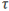is the diffusive stress tensor,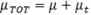is the total viscosity (molecular plus turbulent), r is the radius vector,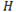is the enthalpy,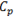is the specific heat, q is the heat flux vector,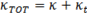is the total conductivity (molecular plus turbulent),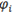, i=1, ...,nSpecs are the species variables,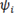is the diffusive flux for species i,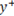is the turbulencevalue at the first node away from the surface,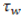is the wall shear stress,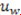is the normalized velocity vector at the first point off the wall, t is the unit vector parallel to the flow, h is the surface film coefficient extracted from the self-similarity solution near the edge of the boundary layer,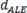is the mesh displacement,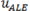is the mesh velocity, k is the turbulent kinetic energy, ω is the turbulent eddy frequency, σ is the viscoelastic stress tensor and x is the coordinate vector.

By default, the moment output variable is computed about the global origin (0,0,0). This moment can be transformed to any desired location by using the -moment_center option of AcuTrans. For example, to evaluate the moment on a surface named "wall surface" about the point 1,0,0, the following command is used:
acuTrans -pb channel -osi -osis "wall surface" -osiv moment -mc 1.0,0.0,0.0
For moving mesh applications where the body of interest is moving, it is possible to compute the moment about a non-stationary point. For example, it may be desirable to compute the moment about the center of gravity of a rigid body. To accomplish this, the initial center point of the body is specified using the -moment option and the motion of the point is determined by referring to the MESH_MOTION command that controls the position of the body using the -moment_center_mesh_motion option. For example, to compute the moment about the center of gravity of an object undergoing rigid body motion, the following command is used:
acuTrans -pb movingMesh -osi -osis "rigid walls" -osiv moment -mc 0.5,0.5,0.0 -mcmm "my rigid body"
surface_statistics_output
The statistics of a given surface output set are written as a two dimensional table in an output file named problem_srf.oss, where srf is a surface ID, starting from one, corresponding to each SURFACE_OUTPUT command in the order given in the input file. The rows of the tables correspond to all the available time steps. The columns correspond to the translated data, in the order given in the surface_statistics_output_vars table. Each data type occupies one or more columns, as given in the Fields column of the above table. For example, assume that "wall -surface" is the second SURFACE_OUTPUT command in the input file, then
acuTrans -pb channel -oss -osss "wall surface" -ossv step,velocity
creates the file channel_srf2.oss containing time step numbers and the surface statistics of velocity, for a total of ten columns. The definitions of the surface statistic output variables are given by:
Variable
Definition
min_velocity
min(uj)j=1,...,num. surface nodes
min_pressure
min(pj)j=1,...,num. surface nodes
min_total_pressure
min(PTOTj)j=1,...,num. surface nodes
min_temperature
min(Tj)j=1,...,num. surface nodes
min_total_temperature
$\mathrm{min}{\left({T}_{TOT\text{\hspace{0.17em}}j}\right)}_{j=1,...num}\text{\hspace{0.17em}}$ surface nodes
min_species
min(φj)j=1,...,num. surface nodes
minimum_field
min(Fj)j=1,...,num. surface nodes
min_eddy_viscosity
min(μtj)j=1,...,num. surface nodes
min_kinetic_energy
min(kj)j=1,...,num. surface nodes
min_eddy_frequency
$\mathrm{min}\left(\frac{1}{\sqrt{{\omega }_{j}}}\right)$ j=1,...,num. surface nodes
min_sqrt_eddy_period
$\mathrm{min}\left(\frac{1}{\sqrt{{\omega }_{j}}}\right)$ j=1,...,num. surface nodes
min_dissipation_rate
min(εj)j=1,...,num. surface nodes
min_intermittency
min( $\gamma$ j)j=1,...,num. surface nodes
min_transition_re_theta
min(Reθjj=1,...,num. surface nodes
min_mesh_displacement
min(dALEj)j=1,...,num. surface nodes
min_mesh_velocity
min(uALEj)j=1,...,num. surface nodes
min_surface_y_plus
min(y+j)j=1,...,num. surface nodes
min_surface_film_coefficient
min(hj)j=1,...,num. surface nodes
min_wall_shear_stress
min(τwuwj)j=1,...,num. surface nodes
min_density
min( $\rho$ j)j=1,...,num. surface nodes
min_viscoelastic_stress
min( $\sigma$ j)j=1,...,num. surface nodes
max_velocity
max(uj)j=1,...,num. surface nodes
max_pressure
max(pj)j=1,...,num. surface nodes
max_total_pressure
max(PTOTj)j=1,...,num. surface nodes
max_temperature
max(Tj)j=1,...,num. surface nodes
max_total_temperature
$\mathrm{max}{\left({T}_{TOT\text{\hspace{0.17em}}j}\right)}_{j=1,...num}\text{\hspace{0.17em}}$ surface nodes
max_species
max(φj)j=1,...,num. surface nodes
max_field
max(Fj)j=1,...,num. surface nodes
max_eddy_viscosity
max(μtj)j=1,...,num. surface nodes
max_kinetic_energy
max(kj)j=1,...,num. surface nodes
max_eddy_frequency
max( $\omega$ j)j=1,...,num. surface nodes
max_sqrt_eddy_period
$\mathrm{max}\left(\frac{1}{\sqrt{{\omega }_{j}}}\right)$ j=1,...,num. surface nodes
max_dissipation_rate
max(εj)j=1,...,num. surface nodes
max_intermittency
max( $\gamma$ j)j=1,...,num. surface nodes
max_transition_re_theta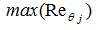j=1,...,num. surface nodes
max_mesh_displacement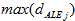j=1,...,num. surface nodes
max_mesh_velocity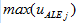j=1,...,num. surface nodes
max_surface_y_plus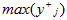j=1,...,num. surface nodes
max_surface_film_coefficientj=1,...,num. surface nodes
max_wall_shear_stress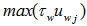j=1,...,num. surface nodes
max_density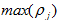j=1,...,num. surface nodes
max_viscoelastic_stress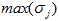j=1,...,num. surface nodes
std_velocity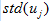j=1,...,num. surface nodes
std_pressure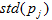j=1,...,num. surface nodes
std_total_pressure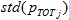j=1,...,num. surface nodes
std_temperature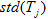j=1,...,num. surface nodes
std_total_temperature
$\text{std}{\left({T}_{TOT\text{\hspace{0.17em}}j}\right)}_{j=1,...num}\text{\hspace{0.17em}}$ surface nodes
std_species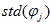j=1,...,num. surface nodes
std_field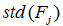j=1,...,num. surface nodes
std_eddy_viscosity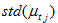j=1,...,num. surface nodes
std_kinetic_energy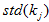j=1,...,num. surface nodes
std_eddy_frequency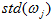j=1,...,num. surface nodes
std_sqrt_eddy_period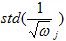j=1,...,num. surface nodes
std_dissipation_rate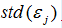j=1,...,num. surface nodes
std_intermittency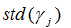j=1,...,num. surface nodes
std_transition_re_theta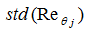j=1,...,num. surface nodes
std_mesh_displacement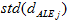j=1,...,num. surface nodes
std_mesh_velocity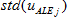j=1,...,num. surface nodes
std_surface_y_plus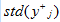j=1,...,num. surface nodes
std_surface_film_coefficient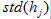j=1,...,num. surface nodes
std_wall_shear_stress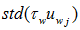j=1,...,num. surface nodes
std_density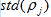j=1,...,num. surface nodes
std_viscoelastic_stress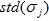j=1,...,num. surface nodes
uni_velocity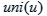uni_pressure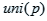uni_total_pressure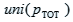uni_temperature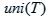uni_total_temperature
$uni\left({T}_{TOT\text{\hspace{0.17em}}}\right)$
uni_species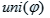uni_field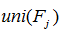j=1,...,num. surface nodes
uni_eddy_viscosity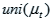uni_kinetic_energy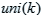uni_eddy_frequency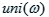uni_sqrt_eddy_period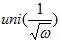uni_dissipation_rate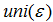uni_intermittency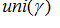uni_transition_re_theta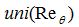uni_mesh_displacement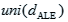uni_mesh_velocity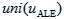uni_surface_y_plus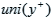uni_surface_film_coefficient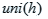uni_wall_shear_stress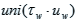uni_density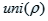uni_viscoelastic_stress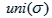where the statistics are computed over the nodes defined by the corresponding SURFACE_OUTPUT command.

The uniformity index of a variable x is defined as follows:(1)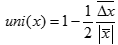where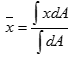and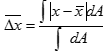output_radiation_surface

The nodal values of a given radiation surface set and a given time step are written as a two dimensional table in an output file named problem_srfsrf_stepstep.orf, where srf is a surface ID, starting from one, corresponding to each RADIATION_SURFACE command in the order given in the input file. The rows of the tables correspond to all the nodes of the surface, numerically sorted. The columns correspond to the translated data, in the order given in the output_radiation_surface_vars table. Each data type occupies one or more columns, as given in the Fields column of the above table. For example, assume that "wall surface" is the second RADIATION_SURFACE command in the input file, then

acuTrans -pb channel -orf -orfs "wall surface" -ts 10

creates the file channel_srf2_step10.orf containing all the output data available for that radiation surface.

output_radiation_integral

The integrated results of a given radiation surface set are written as a two dimensional table in an output file named problem_srfsrf.ori, where srf is a surface ID, starting from one, corresponding to each RADIATION_SURFACE command in the order given in the input file. The rows of the tables correspond to all the available time steps. The columns correspond to the translated data, in the order given in the output_radiation_integral_vars table. Each data type occupies one or more columns, as given in the Fields column of the above table. For example, assume that "wall surface" is the second RADIATION_SURFACE command in the input file, then

acuTrans -pb channel -ori -oris "wall surface" -oriv step,heat

creates the file channel_srf2.ori containing time step numbers and the surface integral of radiation part of the heat flux, for a total of two columns.

output_solar_radiation_surface

The nodal values of a given solar radiation surface set and a given time step are written as a two dimensional table in an output file named problem_srfsrf_stepstep.oqf, where srf is a surface ID, starting from one, corresponding to each SOLAR_RADIATION_SURFACE command in the order given in the input file. The rows of the tables correspond to all the nodes of the surface, numerically sorted. The columns correspond to the translated data, in the order given in the output_solar_radiation_surface_vars table. Each data type occupies one or more columns, as given in the Fields column of the above table. For example, assume that "wall surface" is the second SOLAR_RADIATION_SURFACE command in the input file, then

acuTrans -pb channel -oqf -oqfs "wall surface" -ts 10

creates the file channel_srf2_step10.oqf containing all the output data available for that solar radiation surface.

output_solar_radiation_integral

The integrated results of a given solar radiation surface set are written as a two dimensional table in an output file named problem_srfsrf.oqi, where srf is a surface ID, starting from one, corresponding to each SOLAR_RADIATION_SURFACE command in the order given in the input file. The rows of the tables correspond to all the available time steps. The columns correspond to the translated data, in the order given in the output_solar_radiation_integral_vars table. Each data type occupies one or more columns, as given in the Fields column of the above table. For example, assume that "wall surface" is the second SOLAR_RADIATION_SURFACE command in the input file, then

acuTrans -pb channel -oqi -oqis "wall surface" -oqiv step,heat

creates the file channel_srf2.oqi containing time step numbers and the surface integral of solar radiation part of the heat flux, for a total of two columns.

element_integral_output

The integrated results of a given element output set are written as a two dimensional table in an output file named problem_elemelem.oei, where elem is an element output ID, starting from one, corresponding to each ELEMENT_OUTPUT command in the order given in the input file. The rows of the tables correspond to all the available time steps. The columns correspond to the translated data, in the order given in the element_integral_output_vars table. Each data type occupies one or more columns, as given in the Fields column of the above table. For example, assume that "sample one" is the second ELEMENT_OUTPUT command in the input file, then

acuTrans -pb channel -oei -oeis "sample one" -oeiv time,temp

creates the file channel_elem2.oei containing run time and the spatially averaged temperature, for a total of two columns.

time_history_output

The nodal time history of a given time history output set and node is written as a two dimensional table in an output file named problem_setset_nodenode.oth, where set is a set ID, starting from one, corresponding to each TIME_HISTORY_OUTPUT command in the order given in the input file and node is the node number of the set. The rows of the tables correspond to all the available time steps. The columns correspond to the translated data, in the order given in the time_history_output_vars table. Each data type occupies one or more columns, as given in the Fields column of the above table. For example, assume that nodes 1234 and 5678 appear in the second TIME_HISTORY_OUTPUT command in the input file, then

acuTrans -pb channel -oth -othn 1234,5678 -othv time,vel

creates the files channel_set2_node1234.oth and channel_set2_node5678.oth containing both the run time and nodal velocities of nodes 1234 and 5678, respectively.

fan_component_output

The integrated results of a given fan component set are written as a two dimensional table in an output file named problem_fanfan.ofc, where the second fan is a fan ID, starting from one, corresponding to each FAN_COMPONENT command in the order given in the input file. The rows of the tables correspond to all the available time steps. The columns correspond to the translated data, in the order given in the fan_component_output_vars table. Each data type occupies one or more columns, as given in the Fields column of the above table. For example, assume that "engine fan" is the second FAN_COMPONENT command in the input file, then

acuTrans -pb vehicle -ofc -ofcs "engine fan" -ofcv step,mass

creates the file vehicle_fan2.ofc containing time step numbers and the mass flux across the fan surface, for a total of two columns.

heat_exchanger_component_output

The integrated results of a given heat exchanger component set are given as a two dimensional table in an output file named problem_hechec.ohc, where hec is a heat exchanger component ID, starting from one, corresponding to each HEAT_EXCHANGER_COMPONENT command in the order given in the input file. The rows of the tables correspond to all the available time steps. The columns correspond to the translated data, in the order given in the heat_exchanger_component_output table. Each data type occupies one or more columns, as given in the Fields column of the above table. For example, assume that "radiator" is the second HEAT_EXCHANGER_COMPONENT command in the input file, then

acuTrans -pb vehicle -ohc -ohcs radiator -ohcv step,temp,cool_temp

creates the file vehicle_hec2.ohc containing time step numbers, area-averaged inlet air temperature, and coolant top water temperature, for a total of three columns.

aero_acoustic_output

The computational aero-acoustic data of a given CAA output set and a given time step are written as a two dimensional table in an output file named problem_setset_stepstep.oaa, where set is a set ID, starting from one, corresponding to each CAA_OUTPUT command in the order given in the input file. The rows in the table correspond to the sample points, written in the same order given by the corresponding CAA_OUTPUT command in the input file. The columns correspond to the translated data, in the order given in the aero_acoustic_output_vars table. Each data type occupies one or more columns, as given in the Fields column of the above table. For example, assume that "CAA 2" is the second CAA_OUTPUT command in the input file, then

acuTrans -pb channel -oaa -oaas "CAA 2" -oaav crd,lighthill_stress -ts 10

creates the file channel_set2_step10.oaa containing the sample point coordinates and Lighthill stress at step 10 with a total of nine columns. Note that it is not necessary for each set to have the same variables available. For example, a common strategy requires the divergence of the lighthill stress in the volume and the normal component of the divergence of the total stress on the permeable surfaces.

output_nodal_residual
The nodal residual output of a given time step is written as a two dimensional table in an output file named problem_stepstep.onr. The rows in the table correspond to the coordinates node numbers, written in the same order given by the COORDINATE command in the input file. The columns correspond to the translated data, in the order given in the output_nodal_residual_vars table. Each data type occupies one or more columns, as given in the Fields column of the above table. The following example,
acuTrans -pb channel -onr -onrv vel,temp -ts 10

creates the file channel_step10.onr, which has a total of four columns containing the momentum and heat equation residuals.

output_error_estimator

The error estimator output of a given time step is written as a two dimensional table in an output file named problem_stepstep.oee. The rows in the table correspond to the coordinates node numbers, written in the same order given by the COORDINATE command in the input file. The columns correspond to the translated data, in the order given in the output_error_estimator_vars table. Each data type occupies one or more columns, as given in the Fields column of the above table. The following example,

acuTrans -pb channel -oee -oeev vel,temp -ts 10

creates the file channel_step10.oee, which has a total of four columns containing an error estimate for the momentum and heat equations.

time_average_error_estimator

The time-averaged error estimator output of a given time step is written as a two dimensional table in an output file named problem_stepstep.oae. The rows in the table correspond to the coordinates node numbers, written in the same order given by the COORDINATE command in the input file. The columns correspond to the translated data, in the order given in the time_average_error_estimator_vars table. Each data type occupies one or more columns, as given in the Fields column of the above table. The following example,

acuTrans -pb channel -oae -oaev vel,temp -ts 10

creates the file channel_step10.oae, which has a total of four columns containing a time-averaged error estimate for the momentum and heat equations.

## Stats format

For stats format, AcuTrans computes and prints the minimum, maximum and average of each data field. For nodal_output, running_average_output,time_average_output, derived_quantity_output, surface_output, output_radiation_surface, output_solar_radiation_surface, aero_acoustic_output, output_nodal_residual, output_error_estimator, and time_average_error_estimator the statistics are gathered over the number of nodes; and for surface_integral_output, surface_statistics_output, output_radiation_integral,output_solar_radiation_integral, element_integral_output,time_history_output, fan_component_output, and heat_exchanger_component_output the statistics are gathered over the available time steps. For example, the channel problem

acuTrans -verbose 1 -osf -to stats
outputs the following to the screen:
acuTrans:  Problem = channel
acuTrans:  Run = 1
acuTrans:  Translation format = stats
acuTrans:  Translate to stats = surface output
acuTrans:  Surface name = wall surface
acuTrans:  Surface ID = 0
acuTrans:  Surface output vars = node_id,mass_flux,momentum_flux,traction
acuTrans:  Process time step = 5
acuTrans:  Variable
acuTrans:  mass_flux
acuTrans:  x_momentum_flux
acuTrans:  y_momentum_flux
acuTrans:  z_momentum_flux
acuTrans:  x_traction
acuTrans:  y_traction
acuTrans:  z_traction
acuTrans: Total CPU/Elapse time = 2.000000e-02 3.431511e-02 Sec
acuTrans: Total Memory Usage = 3.222275e-02 Mbytes

When computing statistics of variables not defined everywhere, usually those associated with extended_nodal_output, see nodal_output above, it is often useful to also specify ignore_zeros. The statistics will then be taken only over those nodes with non-zero values.

## CGNS Format

For CGNS format, AcuTrans translates the output data to the (CFD General Notation System) CGNS database file format using version 3.2.1 of the CGNS library. See www.cgns.org. The mesh and nodal_output results of the specified time steps are written in a file named problem.cgns. For example, the channel problem
acuTrans -out -to cgns

creates the database file channel.cgns.

## FieldView Format

For FieldView format, AcuTrans translates the output data to a FieldView unstructured binary file, formats 2.4 or 2.7. FieldView is a visualization package distributed by Intelligent Light. See www.ilight.com. If fieldview_options option is set to classical, the nodal_output results of each time step along with the nodal coordinates, element connectivity and all surface definitions are written to files named problem_stepstep.fv. The surface definitions of each ELEMENT_BOUNDARY_CONDITION command are written under the FieldView boundary surface name "EBC: name", where name is the name of the set. Similarly the surface definitions of each SIMPLE_BOUNDARY_CONDITION, PARTICLE_SURFACE, SURFACE_OUTPUT, and TURBULENCE_WALL command are written under the FieldView boundary surface name "CBC: name", "PSF: name", "OSF: name", and "TWS: name", respectively. A FieldView region file named problem_stepstep.fv.fvreg is also created for each step that is translated. For example, the channel problem

acuTrans -out -to fieldview

creates the file channel_step000005.fv, which contains the nodal coordinates, element connectivity, boundary surfaces "EBC: outflow" and "OSF: wall surface", and the nodal values of velocity and pressure. It also creates the file channel_step000005.fv.fvreg.

When fieldview_options is set to split, FieldView split file format, version 2.7, is used. Here the mesh is written to a file named problem_mesh.fv, while the nodal solution of each time step is written to the file problem_step.fv. A FieldView region file named problem_mesh.fv.fvreg is also created. For example, the channel problem

acuTrans -out -to fieldview -fvopt split

creates the files channel_mesh.fv, channel_step000005.fv, and channel_mesh.fv.fvreg. This option eliminates duplications in mesh output. However, you need FieldView 8.2 or later to read these files.

The FieldView region file contains information that helps FieldView to decompose the information in the .fv files. It is not required in general but it is necessary for parallel visualization. The parameter fieldview_region controls how regions are created. A value of single creates a single region, medium creates one region per medium, fluid, solid, shell, and none, element_set creates one region per element set, and domain creates one region per original subdomain.

For simulations that include mesh motion, there are three options for how the position of the mesh is written to disk. The first option is to write the undeformed coordinates. This enables visualization of the solution with the nodes in the original position:
acuTrans -out -to fieldview -no_ale
The second option is to write the deformed position of the nodes at each time step, and have their location correspond to the position at the end of the time step. This is the result that AcuTrans will produce based on the default settings:
acuTrans -out -to fieldview -ale -deformed_crd_type endstep
The third option is to write the deformed position of the nodes at each time step, and have their location correspond to the position at the mid point of the time step. This option is useful for investigating the continuity of the flow across non-conformal interfaces.
acuTrans -out -to fieldview -ale -deformed_crd_type midstep

## H3D Format

For H3D format, AcuTrans translates the output data to H3D file format. H3D, or Hyper3D, is a compressed binary file format designed to efficiently store Finite Element model and corresponding result data. H3D files can be visualized by HyperView, a full-featured post-processor, and HyperView Player, a free post-processor viewer.

If the h3d_options option is set to multi, the nodal coordinates, element connectivity, and all surface definitions are written to a file named problem.h3d. The nodal_output results of each time step are written to separate files named problem_step.h3d. For example, the channel problem

acuTrans -out -to h3d

creates the file channel.h3d, which contains the nodal coordinates, element connectivity, and boundary surfaces.In addition, it creates the file channel_0005.h3d, which contains the nodal values of velocity and pressure.

When h3d_options is set to single, H3D single file format is used. Here both model and result data are written in a single file named problem.h3d. For example, the channel problem

acuTrans -out -to h3d -h3dopt single

creates the file channel.h3d, which contains the nodal coordinates, element connectivity, boundary surfaces, and the nodal values of velocity and pressure.

## I-deas Format

For I-deas format, AcuTrans translates the output data to the I-deas Universal file format. This is a file format created by Structural Dynamics Research Corporation. The nodal_output results of each time step are written in a file named problem_resstep.unv. The nodal coordinates and element connectivity of the problem are written in a file named problem_mesh.unv, if option mesh_output is set. For example, the channel problem

acuTrans -mesh -out -to ideas

creates the files channel_res5.unv and channel_mesh.unv.

## Spectrum Format

For spectrum format, AcuTrans translates the output data to the Spectrum Visualizer compressed ASCII file format. Spectrum Visualizer is a visualization package created by Centric Engineering Systems, Inc., now part of ANSYS, Inc. The nodal_output and surface_output results of each time step are written in a file named problem_resstep.vis.Z. The nodal coordinates, element connectivity, and surface definitions of the problem are written in a file named problem_mesh.vis.Z, if option mesh_output is set. The surface definitions are written as two dimensional elements and their corresponding surface output is written as the projected nodal values of these elements. For example, the channel problem

acuTrans -mesh -out -osf -to spectrum

creates the files channel_res5.vis.Z and channel_mesh.vis.Z.

## EnSight Format

For EnSight format, AcuTrans translates the output data to the EnSight file format. This option creates a number of files. The primary one is problem.case, which references the other files. These other files are all contained in the directory ENSIGHT.DIR. The nodal_output results of each time step are written in files named problem.var.step. The nodal coordinates and element connectivity of the problem are written in a file named problem_mesh.geo, if option mesh_output is set. For example, the channel problem

acuTrans -mesh -out -to ensight
creates the files channel.case, channel_mesh.geo, channel.velocity.000001, and channel.pressure.000001. The file channel.case looks like
FORMAT
type: ensight
GEOMETRY
model: channel_mesh.geo
VARIABLE
vector per node: velocity channel.velocity.******
scalar per node: pressure channel.pressure.******
TIME
time set: 1
number of steps: 1
filename numbers:
1
time values:
0.1

## Actran Format

For Actran format, AcuTrans translates aero-acoustic output data to the HDF5 file format. Only aero_acoustic_output is supported. For example:
acuTrans -oaa -oaas "CAA 2" -to actran -mesh \
-oaav vel,pres,norm_div_total_stress,div_total_stress,div_lighthill_stress

creates the files coordiantes.hdf and res_step.hdf which are HDF5 files to be read by ActranLA from Free Field Technologies S.A. to perform acoustic propagation calculations. The variables listed in this example are the only ones used by ActranLA so they are the only ones translated. All others are ignored.